• 最近看到了一个3D旋转的动画，就想着自己去实现以下。那么，接下来就通过这边文章记录以下学习过程，慢慢深入了解以下3D旋转。一、如何旋转每个View都在系统的坐标系中，就手机屏幕来说，左上角为 (0 , 0)，向右横向...
最近看到了一个3D旋转的动画，就想着自己去实现以下。那么，接下来就通过这边文章记录以下学习过程，慢慢深入了解以下3D旋转。

一、如何旋转

每个View都在系统的坐标系中，就手机屏幕来说，左上角为 (0 , 0)，向右横向的为X轴正方向，向下纵向的为Y轴正方向，垂直于手机屏幕的方向既Z轴方向。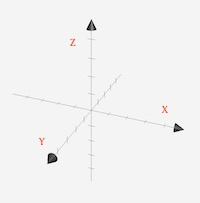所以Z轴的旋转属于平面上的旋转，实现3D效果的前提就是有X轴或者Y轴的参与。

二、沿着Y轴旋转

先看一下CATransform3DMakeRotation的定义：

CA_EXTERN CATransform3D CATransform3DMakeRotation (CGFloat angle, CGFloat x, CGFloat y, CGFloat z)

angle：角度    x,y,z分别代表是三个方向的坐标

按照这个思路，于是我写下如下的代码：

CATransform3D rotate = CATransform3DMakeRotation(M_PI/6, 0, 1, 0);

self.imageView.layer.transform = rotate;


运行之后可以发现，imageView 并没有出现3D效果，只是单纯的在Y轴缩短了一些。为何会出现这样的效果？

查资料后发现，原来在CALayer的显示系统中，默认使用的是正交投影，没有远小近大效果，所以我们对 imageView 的旋转操作，只能造成Y轴上的缩放。而无法观察到3D 的效果。

其实这个时候 imageView 已经沿着Y轴旋转了 M_PI/6 ，但只是我们无法直接观察到这种效果。

三、添加3D效果

这时候我们需要用到透视投影。

先看每个矩阵里数字代表的含义：

 struct CATransform3D
{

CGFloat m11（x缩放）, m12（y切变）, m13（旋转）, m14（）;

CGFloat m21（x切变）, m22（y缩放）, m23（）, m24（）;

CGFloat m31（旋转）, m32（）, m33（）, m34（透视效果）;

CGFloat m41（x平移）, m42（y平移）, m43（z平移）, m44（）;

};

我们所需要的正是 m34 。


构造一个旋转矩阵

CATransform3D rotate = CATransform3DMakeRotation(M_PI/6, 0, 1, 0);

构造一个透视矩阵

其中：M34属性 控制透视效果

正的值向下（左）方往里缩放  负的值上（右）方往里缩放

disZ  控制透视的强度  数字越小 深度越大 拉伸效果越明显

CGFloat disZ = 200;

CATransform3D scale = CATransform3DIdentity;

scale.m34 = -1.0f/disZ; 

然后把两个矩阵相乘  旋转矩阵*透视矩阵

CATransform3D transform = CATransform3DConcat(rotate, scale);    

把最后的结果赋给 imageView 的 layer 的 transform 。

self.imageView.layer.transform = transform;

运行起来，具有3D效果的一张图就展示在我们的眼前了，啧啧，是不是挺简单。

四、控制旋转中心轴

如果通过角度的递增，给我们的3D旋转加一个动画，我们就会明显的看到，默认的以平行于Y轴的  imageView 的中心轴进行旋转。那么如果让我们 imageView 以自己的左边线进行旋转呢？

其实也很简单，旋转是以layer的锚点为中心进行旋转的。默认是 (0.5 , 0.5) 。我们只需要改变锚点的位置就好了。

image.layer.anchorPoint = CGPointMake(0, 0.5); 
展开全文transform 透视变换
• 3D旋转与图片裁剪功能，里面有详细的注释，看一遍就会了，
• 二维图像的显示 矩阵变换 基向量 线性变换 线性变换的复合 平移变换 ...带透视效果的CATransform3D旋转 基于Pan手势的3D旋转控制 总体实现思路 通过向量计算当前手指的移动方向 总结 今...


二维图像的显示
矩阵变换
基向量
线性变换
线性变换的复合
平移变换

齐次坐标
齐次坐标下的点和向量的区别
齐次坐标下的平移变换
齐次坐标下的平行线相交问题

CATransform3D
基于CATransform3D的变换矩阵
3D旋转变换
带透视效果的CATransform3D旋转

基于Pan手势的3D旋转控制
总体实现思路
通过向量计算当前手指的移动方向

总结

今次我们来弄一个好玩的效果，纯粹是好玩，我觉得这个效果应该很少在实际项目中用得到吧（当然不排除一些变态项目）。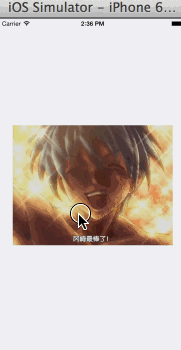当然这个效果运用的核心技术就是CoreAnimation的CATransform3D（还有我的DHVector2D!），那么CATransform3D是个啥玩意呢？不要着急，我们先来了解一点关于计算机图形学的，非常非常基础的东西：矩阵变换和齐次坐标。

计算机图形学是干啥的呢，简单来说的话，因为咱们的电脑（手机）屏幕是二维的，或者说像素点是二维的，但是我们往往要去显示三维的内容（就像上图那样的非常自然的3D变换），那么我应该如何处理这些像素点让它们显示出来的东西看起来是三维的呢？或者说，如何把3维的内容投射到二维平面上去？这就是计算机图形学研究的大致方向，通常一家游戏公司招聘的时候，最首要的要求就是掌握计算机图形学。而计算机图形学的基础是线性代数，怎么样，后悔大学没好好学线性代数了吧？

那么这篇博客我会以尽量简短的内容帮大家理解iOS中的CATransform3D是如何帮助系统让平面的图像（比如一个普通的CALayer）能够进行看起来是三维的变形的，如果你有线性代数的基础，那么在讲解具体代码之前的那部分关于计算机图形学的内容你读起来应该会比较轻松，如果你没有学过线性代数，那么希望你至少能明白一个二维向量是什么东西，那样也能尽量看明白前面的内容，否则的话，只能直接去代码实现的部分了。当然，您也可以参考我技巧篇的这篇博客来对二维向量有一个大致的理解。

二维图像的显示

在讲CAShapeLayer那一章的时候提到过位图和矢量图，而我们的显示器就是一张大大的位图。显示器通过密集地排布像素点来显示任意的图像。那么既然硬件上是这么玩的，我们的UIView和CALayer在显示的时候肯定也是通过排布像素点来绘制图像的。

绘制信息会通过CPU进行计算，在垂直同步信号（iOS默认是开启垂直同步的，这时屏幕刷新信号就等同于垂直同步信号）到来时再提交给GPU进行渲染，然后GPU渲染好了以后提交给帧缓冲区，最后由显示器从帧缓冲区中获得一帧图像显示到显示器上。所以GPU和屏幕都有各自的刷新率，比如你牛逼的1080，可能GPU每秒能渲染144帧图像，但是你的显示器有点辣鸡，每秒只能绘制60帧图像，那么这之间存在的差异就会导致画面撕裂，这时候就需要垂直同步技术来解决画面撕裂。具体的大家可以去网上搜索学习，这里就不做过多解释了。

总之我们要知道一个视图或者CALayer也是通过像素点来显示的，这些像素点的信息由CPU先计算好然后在屏幕刷新信号到来时提交给GPU，然后按照上面的流程显示到屏幕上。

矩阵变换

学习过线性变换的朋友知道，对一个向量进行线性变换（比如旋转、拉伸、斜切等）只需要乘以一个对应的变换矩阵即可。那么为什么乘以这个矩阵就可以得到变换后的向量？以及这个矩阵是怎么得来的？矩阵变换的这部分内容（基向量、线性变换、线性变换的复合、平移变换）主要解决这两个问题，如果感兴趣的话可以读一读，只需要你了解二维向量的姿势即可。如果不感兴趣当然可以直接跳到CATransform3D那里去。

既然我们看到的这些二维图像是通过像素点的排列显示出来的，那么计算机如何处理图像的变换（比如旋转、拉伸等）的呢？

图像的显示涉及到像素点，每个像素点有两个属性：坐标和颜色。在图像进行变形的时候，每个像素点对应的颜色是不会改变的，但是大家应该很轻易的能想到，它们的坐标在变化。那么坐标是如何变化的呢？

作为开发者，我们当然希望存在这样一个神奇函数：它接收一个点为输入参数，然后返回一个新的点，这个返回的点就是输入点变换后所在的点。这样我们把图像上所有的点都拿去调这个函数，就可以得到变换后的图像的所有点的坐标了，那么变换后的图像自然就能画出来了。

这个函数存在吗？实际上在线性代数中有一个和它极其相似的函数，其实在线性代数中它不叫函数，叫变换：线性变换。当然你完全可以把它当做函数来看，因为它也是接收参数，返回结果。线性变换处理的对象是向量，它接收一个向量作为输入参数，然后返回变换后的向量，向量和点之间又存在着那么一丝丝微妙的关系，我们当然可以用线性变换作为入口来考虑上面提到的神奇函数。

所以我们先来看点线代和图形学的基础姿势。

基向量

在平面系统中，我们可以定义一对基向量，用它们来表示平面中任意的向量。如何表示呢？通常在平面坐标系中我们取两个坐标轴上的单位向量为基向量（方向沿坐标轴正方向、长度为1）：x轴上的基向量，记作i⃗=(1,0)$\stackrel{\to }{i}=\left(1,0\right)$$\vec{i} = (1,0)$，y轴上的基向量，记作j⃗=(0,1)$\stackrel{\to }{j}=\left(0,1\right)$$\vec{j} = (0,1)$。

定义了基向量以后，该系统中任意的向量都能用它们的线性组合（加法和数量积）来表示。也就是考虑任意一个向量v⃗=(x,y)$\stackrel{\to }{v}=\left(x,y\right)$$\vec{v} = (x,y)$，那么根据向量加法，我们可以构造两个新向量a⃗=(x,0),b⃗=(0,y)，有v⃗=a⃗+b⃗$\stackrel{\to }{a}=\left(x,0\right),\stackrel{\to }{b}=\left(0,y\right)，有\stackrel{\to }{v}=\stackrel{\to }{a}+\stackrel{\to }{b}$\vec{a} = (x,0),\vec{b} = (0,y)，有\vec{v} = \vec{a} + \vec{b}而根据向量数量积，有a⃗=xi⃗,b⃗=yj⃗$\stackrel{\to }{a}=x\stackrel{\to }{i},\stackrel{\to }{b}=y\stackrel{\to }{j}$\vec{a} = x\vec{i},\vec{b} = y\vec{j}所以有

v⃗=a⃗+b⃗=xi⃗+yj⃗$\stackrel{\to }{v}=\stackrel{\to }{a}+\stackrel{\to }{b}=x\stackrel{\to }{i}+y\stackrel{\to }{j}$\vec{v} = \vec{a} + \vec{b} = x\vec{i} + y\vec{j}

所以任意的向量都可以表示为基向量的线性组合，这是非常重要的思想，请牢牢记在脑海中。

实际上基向量我们可以任意去取，你可以在坐标系中任意找两个向量作为基向量，只要这一对基向量线性无关（不共线），那么它们就可以用来表示这个坐标系下所有存在的向量。

我们来简单证明一下：比如我们自己随意取的基向量分别是i⃗=(a,b),j⃗=(c,d)$\stackrel{\to }{i}=\left(a,b\right),\stackrel{\to }{j}=\left(c,d\right)$$\vec{i} = (a,b), \vec{j} = (c,d)$，那么任意向量v⃗=(x,y)$\stackrel{\to }{v}=\left(x,y\right)$$\vec{v} = (x,y)$如何表示呢？同样因为要用基向量的线性组合来表示，我们只考虑加法和数乘。则对于任意的向量v⃗=(x,y)$\stackrel{\to }{v}=\left(x,y\right)$$\vec{v} = (x,y)$，一定存在两个实数n，m$n，m$$n，m$，使得v⃗=ni⃗+mj⃗=(na,nb)+(mc,md)=(na+mc,nb+md)=(x,y)$\stackrel{\to }{v}=n\stackrel{\to }{i}+m\stackrel{\to }{j}=\left(na,nb\right)+\left(mc,md\right)=\left(na+mc,nb+md\right)=\left(x,y\right)$\vec{v} = n\vec{i} + m\vec{j} = (na,nb) + (mc,md) = (na+mc,nb+md) = (x,y)

那么就有方程组：

{xy=na+mc=nb+md$\left\{\begin{array}{rl}x& =na+mc\\ y& =nb+md\end{array}$\left\{
\begin{aligned}
x & = na+mc \\
y & = nb+md
\end{aligned}
\right.

因为i⃗j⃗$\stackrel{\to }{i}\stackrel{\to }{j}$$\vec{i}\vec{j}$线性无关，即ab≠cd$\frac{a}{b}\ne \frac{c}{d}$$\frac{a}{b} \neq \frac{c}{d}$，也就是ad≠bc$ad\ne bc$$ad \neq bc$，那么方程就存在唯一解：

n=dx−cyad−bc,m=ay−bxad−bc$n=\frac{dx-cy}{ad-bc},m=\frac{ay-bx}{ad-bc}$n = \frac{dx-cy}{ad-bc}, m = \frac{ay-bx}{ad-bc}

那么对于任意的向量v⃗=(x,y)$\stackrel{\to }{v}=\left(x,y\right)$$\vec{v} = (x,y)$，和一对基向量i⃗=(a,b),j⃗=(c,d)$\stackrel{\to }{i}=\left(a,b\right),\stackrel{\to }{j}=\left(c,d\right)$$\vec{i} = (a,b),\vec{j} = (c,d)$我们都能找到两个常数n,m$n,m$$n,m$来表示这个向量v⃗=ni⃗+mj⃗=dx−cyad−bci⃗+ay−bxad−bcj⃗$\stackrel{\to }{v}=n\stackrel{\to }{i}+m\stackrel{\to }{j}=\frac{dx-cy}{ad-bc}\stackrel{\to }{i}+\frac{ay-bx}{ad-bc}\stackrel{\to }{j}$\vec{v} = n\vec{i} + m\vec{j} = \frac{dx-cy}{ad-bc}\vec{i} + \frac{ay-bx}{ad-bc}\vec{j}

顺便一提，如果这对基向量线性相关（共线），那么它们就只能表示它们所在的那条直线上的所有向量；而如果它们都是零向量，则它们只能表示零向量。这应该比较好理解。

关于基向量，要记住两点，非常重要：

你可以取平面上任意一对向量作为基向量

如果这对基向量线性无关，则它们可以表示平面上所有向量；如果线性相关，则它们只能表示它们所在的直线上的所有向量；如果它们都为零向量，则它们只能表示零向量。

这对基向量能表示的所有的向量的集合，叫做这对基向量张成的空间。在平面中，如果这对基向量线性无关，则它们张成的空间就是整个平面；如果它们线性相关，则张成的空间就是它们所在的直线；如果它们是零向量，则它们张成的空间就是原点。

上面的结论同样适用于三维空间甚至更高维空间，大家可以尝试自己去推广一下。

线性变换

在线性代数中有一种变换叫做线性变换，CATransform3D中直接提供的三种变换，旋转(rotate)、拉伸(scale)都是线性变换，而平移(translation)则不是线性变换！后面会专门针对平移变换进行讨论。

线性变换是什么呢，当然线性代数书上给出了明确的定义，而我们作为IT人员，我觉得用一个programmer的思维方式来描述它更为合适。

所以现在我们来以programmer的思维来理解线性变换，考虑有一个函数f$f$$f$，这个函数接收一个向量作为输入参数，然后产生一个新的向量作为返回值，我们先来写一段f的伪代码：

vector f(vector v) {
vector x = v进行某些计算后的结果
return x;
}

就相当于，我们有一个向量v⃗$\stackrel{\to }{v}$$\vec{v}$，然后把v⃗$\stackrel{\to }{v}$$\vec{v}$作为参数传入f$f$$f$，然后通过其返回值得到一个新的向量x⃗$\stackrel{\to }{x}$$\vec{x}$：

x⃗=f(v⃗)$\stackrel{\to }{x}=f\left(\stackrel{\to }{v}\right)$\vec{x} = f(\vec{v})

那么函数f$f$$f$就是一个变换。所以变换就是把一个向量映射成另一个向量的过程（比如旋转，一个向量旋转后就变成另一个向量了）。因为这个映射的过程可以是任意的，如果这个映射的过程满足某些条件的话，嘿嘿，那么这个变换就可以叫做线性变换了。

ok，这“某些条件”是哪些条件啊？按照书上对线性的定义，函数需要满足：

f(v⃗+u⃗)=f(v⃗)+f(u⃗)$f\left(\stackrel{\to }{v}+\stackrel{\to }{u}\right)=f\left(\stackrel{\to }{v}\right)+f\left(\stackrel{\to }{u}\right)$f(\vec{v} + \vec{u}) = f(\vec{v}) + f(\vec{u})
f(cv⃗)=cf(v⃗)$f\left(c\stackrel{\to }{v}\right)=cf\left(\stackrel{\to }{v}\right)$f(c\vec{v}) = cf(\vec{v})

则该函数所代表的变换就是线性的，即可加性和等比例（一阶齐次）。

注意上面两个式子中的向量指的任意向量，c指的任意常数。

而具体地，线性变换如何操作？举个例子，我要实现一个旋转的线性变换，应该对输入向量进行怎样的算法？

还记得我们的基向量吗，这里我不知道数学家们当时的脑回路是怎样的，我只能说这波操作极其风骚。

接下来会出现很多向量相关的等式，但是都是非常基础简单的，不要被吓到，勇敢的去读!（如果比较难理解，大家可以先把下面提到的所有“线性变换”暂时理解成“把一个向量沿原点进行旋转”）

我们知道，平面上任意一个向量都能用基向量的线性组合来表示。我们首先定义一对基向量：
i⃗=(1,0),j⃗=(0,1)$\stackrel{\to }{i}=\left(1,0\right),\stackrel{\to }{j}=\left(0,1\right)$\vec{i} = (1,0),\vec{j} = (0,1)

如果有任意线性变换L$L$$L$，将它作用于任意向量v⃗=(x,y)$\stackrel{\to }{v}=\left(x,y\right)$$\vec{v}=(x,y)$，即L(v⃗)$L\left(\stackrel{\to }{v}\right)$$L(\vec{v})$，等同于L(xi⃗+yj⃗)$L\left(x\stackrel{\to }{i}+y\stackrel{\to }{j}\right)$$L(x\vec{i}+y\vec{j})$

由线性变换的性质：

L(v⃗)=L(xi⃗+yj⃗)=L(xi⃗)+L(yj⃗)=xL(i⃗)+yL(j⃗)$L\left(\stackrel{\to }{v}\right)=L\left(x\stackrel{\to }{i}+y\stackrel{\to }{j}\right)=L\left(x\stackrel{\to }{i}\right)+L\left(y\stackrel{\to }{j}\right)=xL\left(\stackrel{\to }{i}\right)+yL\left(\stackrel{\to }{j}\right)$L(\vec{v}) = L(x\vec{i}+y\vec{j}) = L(x\vec{i}) + L(y\vec{j}) = xL(\vec{i}) + yL(\vec{j})

我们来关注一下结论：

L(v⃗)=xL(i⃗)+yL(j⃗)$L\left(\stackrel{\to }{v}\right)=xL\left(\stackrel{\to }{i}\right)+yL\left(\stackrel{\to }{j}\right)$L(\vec{v}) = xL(\vec{i}) + yL(\vec{j})

大家看着这个等式，能想到什么呢？我再放一个等式在这大家再对比看一下：

v⃗=xi⃗+yj⃗$\stackrel{\to }{v}=x\stackrel{\to }{i}+y\stackrel{\to }{j}$\vec{v} = x\vec{i} + y\vec{j}

还看不出来？再进一步，我们定义两个新的基向量及输出向量：

u⃗=L(v⃗),k⃗=L(i⃗),l⃗=L(j⃗)$\stackrel{\to }{u}=L\left(\stackrel{\to }{v}\right),\stackrel{\to }{k}=L\left(\stackrel{\to }{i}\right),\stackrel{\to }{l}=L\left(\stackrel{\to }{j}\right)$\vec{u} = L(\vec{v}),\vec{k} = L(\vec{i}),\vec{l} = L(\vec{j})

则有：

u⃗=xk⃗+yl⃗(1)$\begin{array}{}\text{(1)}& \stackrel{\to }{u}=x\stackrel{\to }{k}+y\stackrel{\to }{l}\end{array}$\vec{u} = x\vec{k} + y\vec{l}\tag{1}
v⃗=xi⃗+yj⃗(2)$\begin{array}{}\text{(2)}& \stackrel{\to }{v}=x\stackrel{\to }{i}+y\stackrel{\to }{j}\end{array}$\vec{v} = x\vec{i} + y\vec{j}\tag{2}

这样就非常清晰了：对平面上任意一个向量进行线性变换，相当于把平面上的基向量进行该线性变换，然后用新的基向量来表示变换后的向量。也就是对于任意向量v⃗=(x,y)$\stackrel{\to }{v}=\left(x,y\right)$$\vec{v} = (x,y)$，对它进行线性变换L$L$$L$，就相当于对基向量先进行线性变换L$L$$L$，得到新的基向量k⃗,l⃗$\stackrel{\to }{k},\stackrel{\to }{l}$$\vec{k},\vec{l}$，再用这对新的基向量表示向量xk⃗+yl⃗$x\stackrel{\to }{k}+y\stackrel{\to }{l}$$x\vec{k}+y\vec{l}$就是向量v⃗$\stackrel{\to }{v}$$\vec{v}$进行变换后的向量了。

接下来我们对(1)式进行变形：

L(v⃗)=xk⃗+yl⃗=(x,y)(k⃗l⃗)(3)$\begin{array}{}\text{(3)}& L\left(\stackrel{\to }{v}\right)=x\stackrel{\to }{k}+y\stackrel{\to }{l}=\left(x,y\right)\left(\begin{array}{c}\stackrel{\to }{k}\\ \stackrel{\to }{l}\end{array}\right)\end{array}$L(\vec{v}) = x\vec{k} + y\vec{l} = (x,y)\left(\begin{matrix}\vec{k}\\\vec{l}\end{matrix}\right)\tag{3}

其中k⃗=L(i⃗),l⃗=L(j⃗)$\stackrel{\to }{k}=L\left(\stackrel{\to }{i}\right),\stackrel{\to }{l}=L\left(\stackrel{\to }{j}\right)$$\vec{k} = L(\vec{i}),\vec{l} = L(\vec{j})$。

若经过线性变换后得到新的基向量为k⃗=(a,b),l⃗=(c,d)$\stackrel{\to }{k}=\left(a,b\right),\stackrel{\to }{l}=\left(c,d\right)$$\vec{k} = (a,b),\vec{l} = (c,d)$，那么我们可以构造一个矩阵At=(k⃗l⃗)=(acbd)${A}_{t}=\left(\begin{array}{c}\stackrel{\to }{k}\\ \stackrel{\to }{l}\end{array}\right)=\left(\begin{array}{cc}a& b\\ c& d\end{array}\right)$$A_t=\left(\begin{matrix}\vec{k}\\\vec{l}\end{matrix}\right)=\left(\begin{matrix}a&b\\c&d\end{matrix}\right)$

对(3)式进一步计算：

L(v⃗)=xk⃗+yl⃗=(x,y)(k⃗l⃗)=(x,y)(acbd)=(x,y)At=v⃗At$L\left(\stackrel{\to }{v}\right)=x\stackrel{\to }{k}+y\stackrel{\to }{l}=\left(x,y\right)\left(\begin{array}{c}\stackrel{\to }{k}\\ \stackrel{\to }{l}\end{array}\right)=\left(x,y\right)\left(\begin{array}{cc}a& b\\ c& d\end{array}\right)=\left(x,y\right){A}_{t}=\stackrel{\to }{v}{A}_{t}$L(\vec{v})=x\vec{k}+y\vec{l}=(x,y)\left(\begin{matrix}\vec{k}\\\vec{l}\end{matrix}\right)=(x,y)\left(\begin{matrix}a&b\\c&d\end{matrix}\right) =(x,y)A_t=\vec{v}A_t

只关注一下结果：

L(v⃗)=v⃗At，其中At=(k⃗l⃗)=(acbd)$L\left(\stackrel{\to }{v}\right)=\stackrel{\to }{v}{A}_{t}，其中{A}_{t}=\left(\begin{array}{c}\stackrel{\to }{k}\\ \stackrel{\to }{l}\end{array}\right)=\left(\begin{array}{cc}a& b\\ c& d\end{array}\right)$L(\vec{v}) = \vec{v}A_t，其中A_t=\left(\begin{matrix}\vec{k}\\\vec{l}\end{matrix}\right)=\left(\begin{matrix}a&b\\c&d\end{matrix}\right)

看看我们得到了什么结论：

对向量进行线性变换实际上就是让向量乘以一个矩阵At${A}_{t}$$A_t$，所以最关键的过程就是找到这个矩阵，然后让我们的输入向量乘以该矩阵，就完事了。

这样我们就解决了“为什么线性变换就是向量乘以一个矩阵”的问题，接下来我们来看如何构造这个矩阵。

我们先来把伪代码写一写：

vector linear_transformation(vector v) {
// 找到该变换对应的矩阵
matrix At = (a,b,c,d);
// 输入向量左乘矩阵A
vector u = vAt;

return u;
}

这里我们把矩阵At${A}_{t}$$A_t$叫做变换矩阵。

具体地，变换矩阵At${A}_{t}$$A_t$如何来找呢？举个例子，如果我们的线性变换要让所有输入向量都逆时针旋转θ°$\theta °$$\theta°$，按照我们上面基向量的思想，对于任意的输入向量v⃗=(x,y)$\stackrel{\to }{v}=\left(x,y\right)$$\vec{v} = (x,y)$我们只需让两个基向量i⃗=(1,0)和j⃗=(0,1)$\stackrel{\to }{i}=\left(1,0\right)和\stackrel{\to }{j}=\left(0,1\right)$$\vec{i} = (1,0)和\vec{j} = (0,1)$先逆时针旋转θ°$\theta °$$\theta°$，然后得到两个新的基向量k⃗=(a,b)和l⃗=(c,d)$\stackrel{\to }{k}=\left(a,b\right)和\stackrel{\to }{l}=\left(c,d\right)$$\vec{k} = (a,b)和\vec{l} = (c,d)$，然后得到变换矩阵A=(acbd)$A=\left(\begin{array}{cc}a& b\\ c& d\end{array}\right)$$A=\left(\begin{matrix}a&b\\c&d\end{matrix}\right)$，接下来就是如何求a,b,c,d$a,b,c,d$$a,b,c,d$了。先画个图，如图：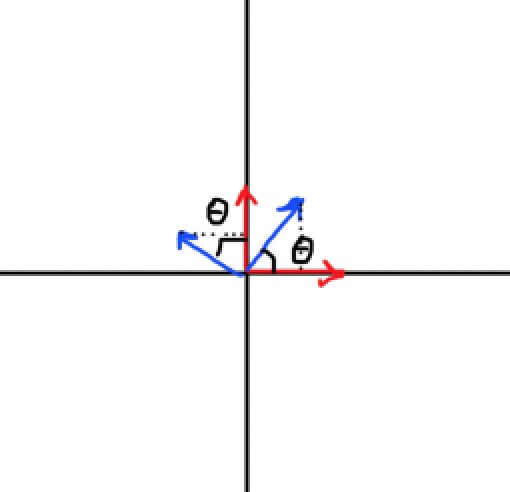在平面直角坐标系中，红色的两个向量为我们的基向量i⃗j⃗$\stackrel{\to }{i}\stackrel{\to }{j}$$\vec{i}\vec{j}$，蓝色的一对向量为基向量逆时针旋转θ°$\theta °$$\theta°$后得到的新的基向量k⃗j⃗$\stackrel{\to }{k}\stackrel{\to }{j}$$\vec{k}\vec{j}$，因为其长度均为1，那么可以得到

k⃗=(cosθ,sinθ)，l⃗=(−sinθ,cosθ)$\stackrel{\to }{k}=\left(\mathrm{cos}\theta ,\mathrm{sin}\theta \right)，\stackrel{\to }{l}=\left(-\mathrm{sin}\theta ,\mathrm{cos}\theta \right)$\vec{k} = (\cos{\theta},\sin{\theta})，\vec{l} = (-\sin{\theta},\cos{\theta})

所以我们得到变换矩阵

A=(cosθ−sinθsinθcosθ)$A=\left(\begin{array}{cc}\mathrm{cos}\theta & \mathrm{sin}\theta \\ -\mathrm{sin}\theta & \mathrm{cos}\theta \end{array}\right)$A=\left(\begin{matrix}\cos{\theta}&\sin{\theta}\\-\sin{\theta}&\cos{\theta}\end{matrix}\right)

注意顺时针旋转的情况有所不同，大家可以自己去求一下顺时针旋转的变换矩阵。

那么对于任意输入向量v⃗=(x,y)$\stackrel{\to }{v}=\left(x,y\right)$$\vec{v} = (x,y)$，其进行线性变换（这里是逆时针旋转θ°$\theta °$$\theta°$）后的输出向量就是

u⃗=L(v⃗)=v⃗At=(x,y)(cosθ−sinθsinθcosθ)=(xcosθ−ysinθ,xsinθ+ycosθ)$\stackrel{\to }{u}=L\left(\stackrel{\to }{v}\right)=\stackrel{\to }{v}{A}_{t}=\left(x,y\right)\left(\begin{array}{cc}\mathrm{cos}\theta & \mathrm{sin}\theta \\ -\mathrm{sin}\theta & \mathrm{cos}\theta \end{array}\right)=\left(x\mathrm{cos}\theta -y\mathrm{sin}\theta ,x\mathrm{sin}\theta +y\mathrm{cos}\theta \right)$\vec{u} = L(\vec{v}) = \vec{v}A_t = (x,y)\left(\begin{matrix}\cos{\theta}&\sin{\theta}\\-\sin{\theta}&\cos{\theta}\end{matrix}\right) = (x\cos{\theta}-y\sin{\theta},x\sin{\theta}+y\cos{\theta})
这个就是向量的旋转公式了，并且我们还解释了为什么任意向量右乘变换矩阵就能得到变换后的新向量(变换矩阵就是由新的基向量构成的，右乘该矩阵的结果刚好等于用这对新的基向量的线性组合来表示输出向量的结果，见(1)式)，并且我们还知道了如何构造变换矩阵（就是求变换后的新的基向量的终点的值是多少，画个图出来就很好理解了）。

大家可以自己尝试求一下顺时针旋转和缩放的变换矩阵。

线性变换的复合

如果我一个向量要作多次变换呢？比如我先旋转，再拉伸要如何操作呢？

那不管怎样，我们先构造一个旋转矩阵Arotate${A}_{rotate}$$A_{rotate}$，一个拉伸矩阵Ascale${A}_{scale}$$A_{scale}$。对于任意的输入向量v⃗$\stackrel{\to }{v}$$\vec{v}$，先旋转，那么得到旋转后的输出向量u⃗=v⃗Arotate$\stackrel{\to }{u}=\stackrel{\to }{v}{A}_{rotate}$$\vec{u} = \vec{v}A_{rotate}$，然后把u⃗$\stackrel{\to }{u}$$\vec{u}$作为拉伸的输入向量，进行拉伸，得到拉伸后的输出向量w⃗=u⃗Ascale$\stackrel{\to }{w}=\stackrel{\to }{u}{A}_{scale}$$\vec{w} = \vec{u}A_{scale}$。也就是说，最先旋转再拉伸的输出向量为：

w⃗=u⃗Ascale=(v⃗Arotate)Ascale$\stackrel{\to }{w}=\stackrel{\to }{u}{A}_{scale}=\left(\stackrel{\to }{v}{A}_{rotate}\right){A}_{scale}$\vec{w} = \vec{u}A_{scale} = (\vec{v}A_{rotate})A_{scale}

而线性运算乘法满足结合律，所以有

w⃗=(v⃗Arotate)Ascale=v⃗(ArotateAscale)=v⃗At$\stackrel{\to }{w}=\left(\stackrel{\to }{v}{A}_{rotate}\right){A}_{scale}=\stackrel{\to }{v}\left({A}_{rotate}{A}_{scale}\right)=\stackrel{\to }{v}{A}_{t}$\vec{w} = (\vec{v}A_{rotate})A_{scale} = \vec{v}(A_{rotate}A_{scale}) = \vec{v}A_t

其中

At=ArotateAscale${A}_{t}={A}_{rotate}{A}_{scale}$A_t = A_{rotate}A_{scale}

也就是说，我们可以将多个变换矩阵复合成一个矩阵，然后输入向量乘以（右乘）这个复合矩阵就相当于进行了多个变换。注意先后顺序，先变换的矩阵要放在乘法的最左边，然后依次右乘接下来的变换矩阵。

平移变换

为什么平移变换不是线性变换呢？在几何上，向量的线性变换的一个必要条件是：线性变换作用于坐标系上所有的点，变换后原点的位置不会发生改变。显然，平移变换后原点的位置会随着平移而发生改变，从几何的角度平移变换也不是线性变换。

顺便一提，另一个必要条件是：变换前处于同一直线上的点，变换后仍要处于同一直线，且变换后它们之间的距离之比与变换前相同（比如变换之前处于同一直线上的三个点A，B，C，变换后分别对应A’，B’，C’，那么A’，B’，C’必须也处于同一直线，且AB/BC = A’B’/B’C’）。你也可以这样理解：变换前所有处于同一直线且等距的点，在变换后也处于同一直线且等距。

我们在齐次坐标中再来具体讨论平移变换如何实现。

齐次坐标

如果大家按住cmd点进CATransform3D的定义里面去就可以看到，CATransform3D实际上是一个四阶方阵（4x4矩阵）。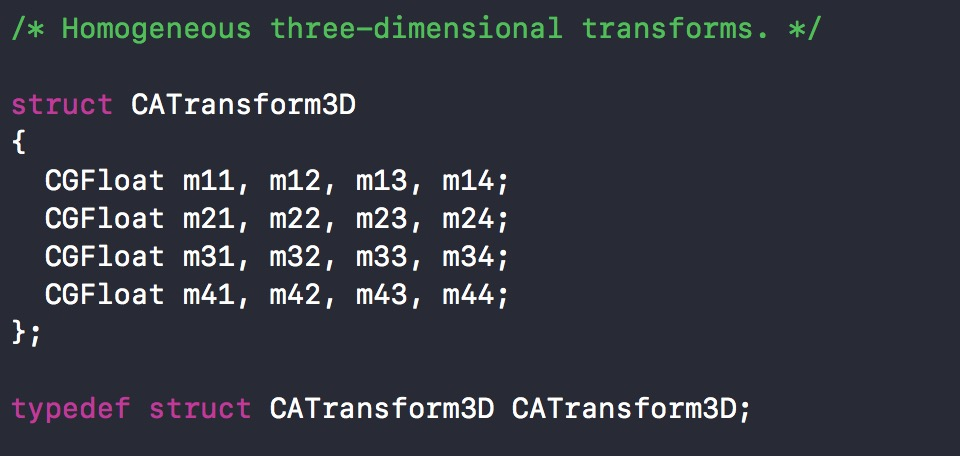备注写着Homogeneous three-dimensional transforms，意思就是齐次3D变换。

为什么要用四维的矩阵表示三维的内容呢，这是使用了齐次坐标，1是数学家们发明出来用来解决欧式几何无法解决的透视问题，在欧式几何（笛卡尔坐标系）中，两条平行线永远不会相交，但是在透视空间中，两条平行线是可以相交于无穷远，如图，火车轨道的两边相汇于无穷远处。2是用来区分点和向量之间的区别。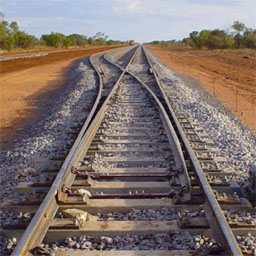齐次坐标下的点和向量的区别

我们先从坐标点和向量坐标表示的区别开始。我们知道平面上一个点可以用一个二元元组来表示：P(x,y)$P\left(x,y\right)$$P(x,y)$，而一个向量也可以如此表示：v⃗=(x,y)$\stackrel{\to }{v}=\left(x,y\right)$$\vec{v} = (x,y)$，这有啥区别呢？

点的坐标是相对于原点的，而向量的坐标是向量终点相对于向量起点的，如果一个向量的起点就是坐标原点，那么此时向量和点在线性变换时没有任何区别。

既然点坐标是相对于原点的，我们可以把点P(x,y)$P\left(x,y\right)$$P(x,y)$看做是原点O(0,0)$O\left(0,0\right)$$O(0,0)$沿着向量v⃗=(x,y)$\stackrel{\to }{v}=\left(x,y\right)$$\vec{v} = (x,y)$平移后的结果。

那么对于平面上的一对基向量i⃗=(1,0),j⃗=(0,1)$\stackrel{\to }{i}=\left(1,0\right),\stackrel{\to }{j}=\left(0,1\right)$$\vec{i} = (1,0),\vec{j} = (0,1)$，我们可以这样表示向量：
v⃗=xi⃗+yj⃗(1)$\begin{array}{}\text{(1)}& \stackrel{\to }{v}=x\stackrel{\to }{i}+y\stackrel{\to }{j}\end{array}$\vec{v} = x\vec{i} + y\vec{j}\tag{1}

而点则看做原点沿着向量的平移，有：

P−O=v⃗$P-O=\stackrel{\to }{v}$P - O = \vec{v}

则可以表示点P$P$$P$：

P(x,y)=xi⃗+yj⃗+O(2)$\begin{array}{}\text{(2)}& P\left(x,y\right)=x\stackrel{\to }{i}+y\stackrel{\to }{j}+O\end{array}$P(x,y) = x\vec{i}+y\vec{j}+O\tag{2}

把(1)式和(2)式写成向量相乘的形式：

v⃗=(x,y)(i⃗j⃗)=(x,y,0)⎛⎝⎜⎜⎜i⃗j⃗O⎞⎠⎟⎟⎟$\stackrel{\to }{v}=\left(x,y\right)\left(\begin{array}{c}\stackrel{\to }{i}\\ \stackrel{\to }{j}\end{array}\right)=\left(x,y,0\right)\left(\begin{array}{c}\stackrel{\to }{i}\\ \stackrel{\to }{j}\\ O\end{array}\right)$\vec{v} = (x,y)\left(\begin{matrix}\vec{i}\\\vec{j}\end{matrix}\right) = (x,y,0)\left(\begin{matrix}\vec{i}\\\vec{j}\\O\end{matrix}\right)

P=(x,y,1)⎛⎝⎜⎜⎜i⃗j⃗O⎞⎠⎟⎟⎟$P=\left(x,y,1\right)\left(\begin{array}{c}\stackrel{\to }{i}\\ \stackrel{\to }{j}\\ O\end{array}\right)$P = (x,y,1)\left(\begin{matrix}\vec{i}\\\vec{j}\\O\end{matrix}\right)

我们看到，一旦我们把原点O$O$$O$作为新的基向量（零向量）来考虑，点和向量有了不同的表示形式。多出的这一维的数字表示和坐标原点的关系，向量是0，表示向量和坐标系原点没有关系，无论你原点在哪都不影响我向量的大小和方向；点是1，表示点坐标是和坐标系原点密切相关的，不同坐标系原点下的P(x,y)$P\left(x,y\right)$$P(x,y)$可能会画出不同的点来。

像这样用N+1维元组来表示N维的点和向量就是齐次坐标表示。

齐次坐标下的平移变换

在此基础上我们再来看平移变换。在线性变换中，点和起点在原点的向量之间是可以直接互相转换的，比如你要让一个点绕着坐标原点旋转，那就可以让一个表达式和点相同的向量绕坐标轴原点旋转（乘以旋转矩阵），然后转换成点即可，其实就是让点(x,y)$\left(x,y\right)$$(x,y)$乘以旋转矩阵就完事了。但是你没法通过乘以某个矩阵让点进行平移：

比如对于平面上任意一点P(x,y)$P\left(x,y\right)$$P(x,y)$，要让它沿着向量v⃗=(a,b)$\stackrel{\to }{v}=\left(a,b\right)$$\vec{v} = (a,b)$进行平移，那么平移后的点就是P′(x+a,y+b)${P}^{\prime }\left(x+a,y+b\right)$$P'(x+a,y+b)$。现在我们来尝试找一个变换矩阵At${A}_{t}$$A_t$，使得：

(x,y)At=(x+a,y+b)$\left(x,y\right){A}_{t}=\left(x+a,y+b\right)$(x,y)A_t = (x+a, y+b)

你会发现永远也找不到这样一个矩阵。现在我们引入齐次坐标，也就是把点用P(x,y,1)$P\left(x,y,1\right)$$P(x,y,1)$来表示，平移后的点就是P′(x+a,y+b,1)${P}^{\prime }\left(x+a,y+b,1\right)$$P'(x+a,y+b,1)$，现在再来尝试找一个变换矩阵At${A}_{t}$$A_t$，使得：

(x,y,1)At=(x+a,y+b,1)$\left(x,y,1\right){A}_{t}=\left(x+a,y+b,1\right)$(x,y,1)A_t = (x+a,y+b,1)

我们就可以找到一个3x3矩阵At=⎛⎝⎜⎜10a01b001⎞⎠⎟⎟${A}_{t}=\left(\begin{array}{ccc}1& 0& 0\\ 0& 1& 0\\ a& b& 1\end{array}\right)$$A_t = \left(\begin{matrix}1&0&0\\0&1&0\\a&b&1\end{matrix}\right)$。显然，为了适配平移变换的问题，我们的旋转和缩放等线性变换也应该放到齐次坐标下来。在二维平面上，对应的旋转矩阵（逆时针旋转）和缩放矩阵的齐次坐标表示分别为：

Arotate=⎛⎝⎜⎜cos−sin0sincos0001⎞⎠⎟⎟,Ascale=⎛⎝⎜⎜sx000sy0001⎞⎠⎟⎟${A}_{rotate}=\left(\begin{array}{ccc}cos& sin& 0\\ -sin& cos& 0\\ 0& 0& 1\end{array}\right),{A}_{scale}=\left(\begin{array}{ccc}sx& 0& 0\\ 0& sy& 0\\ 0& 0& 1\end{array}\right)$A_{rotate} = \left(\begin{matrix}cos&sin&0\\-sin&cos&0\\0&0&1\end{matrix}\right), A_{scale} = \left(\begin{matrix}sx&0&0\\0&sy&0\\0&0&1\end{matrix}\right)

这样在进行变换的复合时，就可以加上平移矩阵了。

这里顺便提一下，图像变换的顺序一定要是先缩放、再旋转、最后平移。这个原因涉及到物体坐标系到世界坐标系的转换，缩放既不改变坐标原点，也不改变坐标轴方向；旋转不改变坐标原点，但会影响坐标轴方向；平移则干脆连坐标原点都变了，所以变换的复合必须按着这个顺序来，不然就会出现奇怪的效果。

这样对于平面上任意的点P(x,y)$P\left(x,y\right)$$P(x,y)$，其在齐次坐标下的表示为P(x,y,1)$P\left(x,y,1\right)$$P(x,y,1)$，然后对它实施各种变换，进行变换后的新点为P′(x′,y′,1)${P}^{\prime }\left({x}^{\prime },{y}^{\prime },1\right)$$P'(x',y',1)$，然后转换回笛卡尔坐标：P′(x′,y′)${P}^{\prime }\left({x}^{\prime },{y}^{\prime }\right)$$P'(x',y')$，这样齐次坐标产生的新的维度就不影响我们在笛卡尔坐标系下的点的表示。

你可能会发现，如果让向量来平移，就算是齐次坐标也无法实现，因为我们找不到这样一个变换矩阵At${A}_{t}$$A_t$，使得

(x,y,0)At=(x+a,y+b,0)$\left(x,y,0\right){A}_{t}=\left(x+a,y+b,0\right)$(x,y,0)A_t = (x+a,y+b,0)

这也印证了我们之前的结论，向量只有方向和大小，没有位置的概念，所以平移变换对一个向量而言是没有意义的。

同时我们可以验证平移变换的可加性，比如考虑一个沿着v⃗=(a,b)$\stackrel{\to }{v}=\left(a,b\right)$$\vec{v} = (a,b)$平移的平移变换：

f(x,y)=(x+a,y+b)$f\left(x,y\right)=\left(x+a,y+b\right)$f(x,y) = (x+a,y+b)

我们验证可加性，对于任意两个点U(ux,uy)和V(vx,vy)$U\left({u}_{x},{u}_{y}\right)和V\left({v}_{x},{v}_{y}\right)$$U(u_x,u_y)和V(v_x,v_y)$，有

f(U+V)=f(ux+vx,uy+vy)=(ux+vx+a,uy+vy+b)$f\left(U+V\right)=f\left({u}_{x}+{v}_{x},{u}_{y}+{v}_{y}\right)=\left({u}_{x}+{v}_{x}+a,{u}_{y}+{v}_{y}+b\right)$f(U+V) = f(u_x+v_x,u_y+v_y) = (u_x+v_x+a, u_y+v_y+b)
f(U)+f(V)=f(ux,uy)+f(vx,vy)=(ux+a,uy+b)+(vx+a,vy+b)=(ux+vx+2a,uy+vy+2b)$f\left(U\right)+f\left(V\right)=f\left({u}_{x},{u}_{y}\right)+f\left({v}_{x},{v}_{y}\right)\phantom{\rule{0ex}{0ex}}=\left({u}_{x}+a,{u}_{y}+b\right)+\left({v}_{x}+a,{v}_{y}+b\right)\phantom{\rule{0ex}{0ex}}=\left({u}_{x}+{v}_{x}+2a,{u}_{y}+{v}_{y}+2b\right)$f(U) + f(V) = f(u_x,u_y)+f(v_x,v_y)
\\=(u_x+a,u_y+b)+(v_x+a,v_y+b)
\\=(u_x+v_x+2a,u_y+v_y+2b)

所以

f(U+V)≠f(U)+f(V)$f\left(U+V\right)\ne f\left(U\right)+f\left(V\right)$f(U+V)\neq f(U)+f(V)

所以平移变换不满足可加性，从线性变换的定义出发也验证了平移变换不是线性变换。

以上就是齐次坐标的第一个作用：解决向量和点之间的区别。

向量没有位置的概念，而点有。而旋转和缩放对于位置是没有关系的，如图，无论你把向量放在哪里，只要向量的表达式不变，那么它旋转后的结果也不变，缩放同理。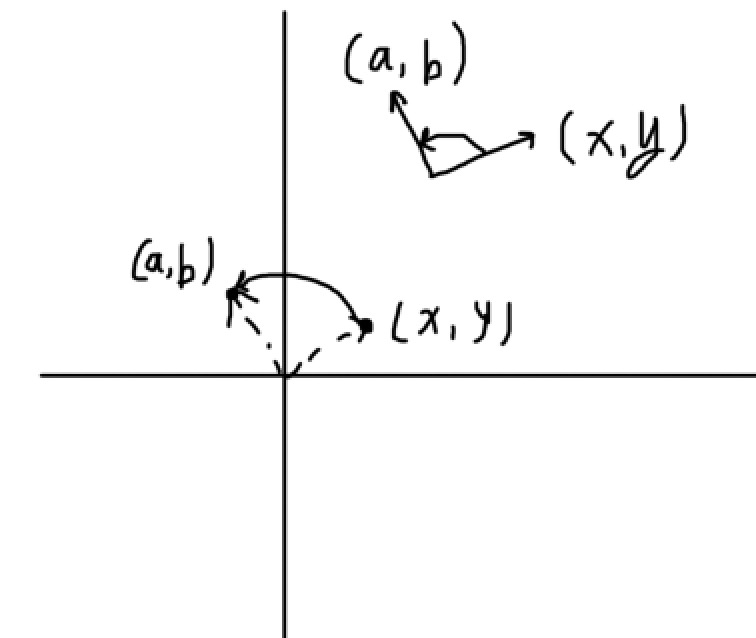那么对于旋转和缩放，点和向量之间的差别（位置）就不起作用了，那么对于这两个变换，点和向量是可以完美互相转换的（相当于对于旋转和缩放而言，它们认为向量和点是同一个东西）。但是我们的变换需求还有平移变换，而平移变换对于没有位置概念的向量而言是没有意义的（N维向量找不到N维平移变换矩阵，也就是用坐标值乘以矩阵来实现变换对于平移变换而言就不存在了），为了适配缩放和旋转（使用矩阵乘法来表示一个变换，这样才能实现变换的复合，将多个变换表示为一个矩阵），我们把N维的点和向量用N+1维的形式来表示，这样对于新的表示下的点P(x,y,1)$P\left(x,y,1\right)$$P(x,y,1)$，就找到了能够用矩阵乘法来表示的平移矩阵，解决了适配的问题。

新的这一维的值如果是1，则表示一个点（因为它和坐标原点相关），如果是0，则表示一个向量（和坐标原点无关），或者表示一个无穷远处的点（无穷远处的点无论你坐标原点在哪，它还是无穷远处的点，所以也和坐标原点无关）。

齐次坐标下的平行线相交问题

最后我们来看，齐次坐标解决透视空间下的两条平行线可以相交的问题。

我们回到齐次坐标对点的表达式P(x,y,1)$P\left(x,y,1\right)$$P(x,y,1)$，不知道有没有朋友在考虑这样一个问题：多的那一位表示和坐标原点“有”关系或者“无”关系，那不就是“非空”与“空”么，也就是“非零”与“零”的关系，那既然有关系用1来表示，为什么不可以用2、3、4…来表示呢？

当然数学家们也想到了这个不严谨的地方，所以他们添加了一条定义：

即当k$k$$k$非零时，所有形如(kx,ky,k)$\left(kx,ky,k\right)$$(kx, ky,k)$的三元组都表示同一个点，比如(x,y,1)$\left(x,y,1\right)$$(x, y, 1)$和(2x,2y,2)$\left(2x,2y,2\right)$$(2x, 2y,2)$就表示同一个点。由此我们就可以引出齐次坐标的定义，即给定一个二维点(x,y)$\left(x,y\right)$$(x, y)$，那么形如(kx,ky,k)$\left(kx,ky,k\right)$$(kx, ky,k)$的所有三元组就都是等价的，它们就是这个点的齐次坐标。对每一个齐次坐标，我们只要把它除以三元组中的第三个数，即可得到原始的二维点坐标。这也就是为什么这玩意叫做“齐次”坐标。

而当k=0$k=0$$k=0$时，因为除数不能为0，也就是点(x,y,0)$\left(x,y,0\right)$$(x,y,0)$是没有意义的，毕竟无论坐标原点在哪，你也无法表示它，这样的点当然就在无穷远的地方了。

好，现在我们开始来解决平行线的相交问题，考虑两条直线：
{Ax+By+C=0Ax+By+D=0$\left\{\begin{array}{r}Ax+By+C=0\\ Ax+By+D=0\end{array}$ \left\{
\begin{aligned}
Ax+By+C=0 \\
Ax+By+D=0
\end{aligned}
\right.

这两条直线是线性相关的（斜率一样，所以是两条平行线）。

在笛卡尔坐标中，如果C≠D$C\ne D$$C\neq D$，那么方程组无解；如果C=D$C=D$$C=D$那它们就是同一条直线了。

现在我们令kx=x′,ky=y′$kx={x}^{\prime },ky={y}^{\prime }$$kx=x',ky=y'$，放到透视空间下来求解：

⎧⎩⎨⎪⎪Ax′k+By′k+C=0Ax′k+By′k+D=0$\left\{\begin{array}{r}A\frac{{x}^{\prime }}{k}+B\frac{{y}^{\prime }}{k}+C=0\\ A\frac{{x}^{\prime }}{k}+B\frac{{y}^{\prime }}{k}+D=0\end{array}$ \left\{
\begin{aligned}
A\frac{x'}{k}+B\frac{y'}{k}+C=0 \\
A\frac{x'}{k}+B\frac{y'}{k}+D=0
\end{aligned}
\right.

整理一下得到

{Ax′+By′+Ck=0Ax′+By′+Dk=0$\left\{\begin{array}{r}A{x}^{\prime }+B{y}^{\prime }+Ck=0\\ A{x}^{\prime }+B{y}^{\prime }+Dk=0\end{array}$ \left\{
\begin{aligned}
Ax'+By'+Ck=0 \\
Ax'+By'+Dk=0
\end{aligned}
\right.

现在我们在C≠D$C\ne D$$C\neq D$的情况下得到一组解(x′,y′,0)$\left({x}^{\prime },{y}^{\prime },0\right)$$(x',y',0)$，也就是说这两条直线相交于无穷远处的一点(x′,y′,0)$\left({x}^{\prime },{y}^{\prime },0\right)$$(x',y',0)$。所以3D图像投射到平面上时，就需要使用齐次坐标来表示点在透视空间下的表示（比如上面的火车轨道那张图，就是平面图形显示3D内容时，如何表示两条平行线的），我们在接下来的CATransform3D就可以看到。

CATransform3D

在开始CATransform3D之前，我们先来回顾一下上面得到的一些结论：

图像是由像素点构成的，要实现图像的各种变形变换，需要一个变换函数，将一个点作为输入参数，输出变换后的点。把构成该图像的所有像素点都拿去调用这个函数，就能实现图像的变形了；
在线性代数中有一种变换叫线性变换，它接收一个向量作为输入参数，输出变形后的向量，比如一个逆时针旋转90°的线性变换，接收任何一个向量，输出的向量就是原向量逆时针旋转90°后的向量；
线性变换的过程实际上就是输入向量乘以某个变换矩阵。我们需要实现的三种基本变换：平移、缩放、旋转中，缩放和旋转是线性变换（满足可加性和一阶齐次），由于矩阵乘法拥有结合律，所以多个线性变换可以通过它们的变换矩阵相乘复合成一个变换矩阵，比如我们可以用缩放矩阵乘以旋转矩阵，得到的结果就是一个描述先缩放再旋转的变换矩阵，输入向量乘以这个矩阵，输出的向量就是先缩放后旋转的结果；
对于平移变换，为了让它也能通过矩阵乘法进行变换的复合，我们发现只有在齐次坐标下才能找到这样一个矩阵，而为了能让平移变换也加入旋转和缩放的复合运算中，旋转和缩放也应该在齐次坐标下来表示，这样它们才能相乘。

有一点要说明一下，以免有同学钻进了牛角尖出不来。并不是因为旋转和缩放是线性变换所以它们才用矩阵乘法来表示。我们描述一个变换，实际上是对向量进行操作，只要输出满足我们的效果就可以了。而之所以要用矩阵乘法来表示旋转和缩放，是因为我们恰好能很方便的通过基向量来找到这样的变换矩阵，并且矩阵乘法满足结合律，多个变换就可以用一个矩阵来表示。平移变换不是线性变换，但是它仍是一个变换，只要是变换，就是考虑输入向量通过某些操作得到输出向量。既然旋转和缩放是用的矩阵乘法，那么为了让平移也能复合进去，我们应该优先考虑平移变换也用矩阵乘法来实现。很明显平移变换最简单的实现就是f(x,y)=(x+a,y+b)$f\left(x,y\right)=\left(x+a,y+b\right)$$f(x,y) = (x+a,y+b)$，这样表示一个点(x,y)$\left(x,y\right)$$(x,y)$向x轴方向平移a个单位，向y轴方向平移b个单位。输入为P(x,y)$P\left(x,y\right)$$P(x,y)$，输出为P′(x+a,y+b)${P}^{\prime }\left(x+a,y+b\right)$$P'(x+a,y+b)$，这样f就是一个完美的平移变换函数。但是如果这样来表示平移变换，就没办法通过矩阵乘法与缩放和旋转进行复合了。所以我们在找这个平移矩阵时，发现只有在齐次坐标下，这个矩阵才存在，于是为了让平移变换用矩阵乘法表示，点和变换矩阵都应该在齐次坐标下，这时旋转和缩放也就应该用齐次坐标来表示了。而线性变换和非线性变换的变换矩阵的区别在于，线性变换的变换矩阵是通过基向量变换后的结果来合成的，因此很好找，而非线性变换，比如平移变换，是“硬算”出来的，它和“用变换后的基向量来表示变换后的向量”毫无关系。再仔细看看基向量那一部分，我们是通过线性变换才有的性质（可加性和一阶齐次）才得出了“用变换后的基向量来表示变换后的向量”的结论，非线性变换可不能这么做。

基于CATransform3D的变换矩阵

从矩阵变换到齐次坐标，为了方便大家理解，我是以二维的情况进行的各种推导，这些结论都可以推广到高维上去。

比如我们接下来就要看的三维变换CATransform3D。在齐次坐标开始的时候我就截了个图，这玩意是一个矩阵，没错，它是一个施加于CALayer的变换矩阵。也就是CALayer上所有的像素点，最终都会乘以这个变换矩阵来实现各种奇奇怪怪的变换效果，比如平移缩放旋转。可能大家都知道，如果要让一个layer进行缩放的变形，一般是这样写的：

// 让一个layer在x轴方向拉伸2倍，在y轴方向拉伸3倍，在z轴方向拉伸1倍。
// 当然一般的layer是没有厚度的概念的，所以z轴的拉伸对layer而言就是没有意义的
layer.transform = CATransform3DMakeScale(2, 3, 1);

这就是改变layer的transform，也就是改变layer的变换矩阵，让layer在绘制的时候，所有的像素点乘以该矩阵来实现变形。同样我们点进CATransform3DMakeScale的注释里面去看

/* Returns a transform that scales by (sx, sy, sz)':
* t' = [sx 0 0 0; 0 sy 0 0; 0 0 sz 0; 0 0 0 1]. */

CA_EXTERN CATransform3D CATransform3DMakeScale (CGFloat sx, CGFloat sy,
CGFloat sz)
CA_AVAILABLE_STARTING (10.5, 2.0, 9.0, 2.0);

这个就是苹果为我们实现好的一个3维齐次坐标下的缩放矩阵：

t′=⎛⎝⎜⎜⎜⎜sx0000sy0000sz00001⎞⎠⎟⎟⎟⎟${t}^{\prime }=\left(\begin{array}{cccc}sx& 0& 0& 0\\ 0& sy& 0& 0\\ 0& 0& sz& 0\\ 0& 0& 0& 1\end{array}\right)$t' = \left(\begin{matrix}sx&0&0&0\\0&sy&0&0\\0&0&sz&0\\0&0&0&1\end{matrix}\right)

这就是三个基向量缩放后的结果组成一个矩阵再转换成齐次坐标：

x轴的基向量i⃗=(1,0,0),y轴的基向量j⃗=(0,1,0),z轴的基向量k⃗=(0,0,1)$x轴的基向量\stackrel{\to }{i}=\left(1,0,0\right),y轴的基向量\stackrel{\to }{j}=\left(0,1,0\right),z轴的基向量\stackrel{\to }{k}=\left(0,0,1\right)$$x轴的基向量\vec{i} = (1,0,0),y轴的基向量\vec{j} = (0,1,0),z轴的基向量\vec{k} = (0,0,1)$

缩放后的基向量分别是：

si→=(sx,0,0),sj→=(0,sy,0),sk→=(0,0,sz)$\stackrel{\to }{si}=\left(sx,0,0\right),\stackrel{\to }{sj}=\left(0,sy,0\right),\stackrel{\to }{sk}=\left(0,0,sz\right)$\vec{si} = (sx,0,0),\vec{sj} = (0,sy,0),\vec{sk} = (0,0,sz)

再组合成非齐次坐标下的变换矩阵：

t=⎛⎝⎜⎜sx000sy000sz⎞⎠⎟⎟$t=\left(\begin{array}{ccc}sx& 0& 0\\ 0& sy& 0\\ 0& 0& sz\end{array}\right)$t = \left(\begin{matrix}sx&0&0\\0&sy&0\\0&0&sz\end{matrix}\right)

最后转换成齐次坐标就是我们的CATransform3DMakeScale的结果，同理大家可以看看旋转矩阵和平移矩阵：

/* Returns a transform that translates by '(tx, ty, tz)':
* t' =  [1 0 0 0; 0 1 0 0; 0 0 1 0; tx ty tz 1]. */

CA_EXTERN CATransform3D CATransform3DMakeTranslation (CGFloat tx,
CGFloat ty, CGFloat tz)
CA_AVAILABLE_STARTING (10.5, 2.0, 9.0, 2.0);

/* Returns a transform that scales by (sx, sy, sz)':
* t' = [sx 0 0 0; 0 sy 0 0; 0 0 sz 0; 0 0 0 1]. */

CA_EXTERN CATransform3D CATransform3DMakeScale (CGFloat sx, CGFloat sy,
CGFloat sz)
CA_AVAILABLE_STARTING (10.5, 2.0, 9.0, 2.0);

/* Returns a transform that rotates by 'angle' radians about the vector
* '(x, y, z)'. If the vector has length zero the identity transform is
* returned. */

CA_EXTERN CATransform3D CATransform3DMakeRotation (CGFloat angle, CGFloat x,
CGFloat y, CGFloat z)
CA_AVAILABLE_STARTING (10.5, 2.0, 9.0, 2.0);

由于赋值会覆盖之前的值，所以使用这三个矩阵赋值的话无论你之前经历了怎样的变换，都会被替换成这一个变换，而不是在之前的变换的基础上进行复合。当然苹果肯定为我们提供了复合的方法：

/* Translate 't' by '(tx, ty, tz)' and return the result:
* t' = translate(tx, ty, tz) * t. */

CA_EXTERN CATransform3D CATransform3DTranslate (CATransform3D t, CGFloat tx,
CGFloat ty, CGFloat tz)
CA_AVAILABLE_STARTING (10.5, 2.0, 9.0, 2.0);

/* Scale 't' by '(sx, sy, sz)' and return the result:
* t' = scale(sx, sy, sz) * t. */

CA_EXTERN CATransform3D CATransform3DScale (CATransform3D t, CGFloat sx,
CGFloat sy, CGFloat sz)
CA_AVAILABLE_STARTING (10.5, 2.0, 9.0, 2.0);

/* Rotate 't' by 'angle' radians about the vector '(x, y, z)' and return
* the result. If the vector has zero length the behavior is undefined:
* t' = rotation(angle, x, y, z) * t. */

CA_EXTERN CATransform3D CATransform3DRotate (CATransform3D t, CGFloat angle,
CGFloat x, CGFloat y, CGFloat z)
CA_AVAILABLE_STARTING (10.5, 2.0, 9.0, 2.0);

/* Concatenate 'b' to 'a' and return the result: t' = a * b. */

CA_EXTERN CATransform3D CATransform3DConcat (CATransform3D a, CATransform3D b)
CA_AVAILABLE_STARTING (10.5, 2.0, 9.0, 2.0);


前三个就是在某个变换（参数t提供的变换）之上进行平移、缩放、旋转（注释就写清楚了，用t乘以新生成了平移、缩放、旋转矩阵来得到复合后的矩阵并返回）。最后一个函数就是把两个参数a和b进行乘法，也就是变换的复合。如果你要自己搞些奇怪的变换，那可以使用这个函数来帮你计算矩阵乘法。

你可以理解成：

// 这是一个数学公式而不是赋值语句
CATransform3DScale(t,sx,sy,sz) = CATransform3DConcat(t,CATransform3DMakeScale(sx,sy,sz))


顺便一提，默认的transform是

/* The identity transform: [1 0 0 0; 0 1 0 0; 0 0 1 0; 0 0 0 1]. */

CA_EXTERN const CATransform3D CATransform3DIdentity

也就是一个单位矩阵，任何点乘以单位矩阵得到点本身，意思就是没有任何变换效果。如果你想从变形后的状态还原，那就用这个矩阵给transform属性赋值就好了。

3D旋转变换

然后我们来看我们这个效果主要用到的，旋转变换。

/* Returns a transform that rotates by 'angle' radians about the vector
* '(x, y, z)'. If the vector has length zero the identity transform is
* returned. */

CA_EXTERN CATransform3D CATransform3DMakeRotation (CGFloat angle, CGFloat x,
CGFloat y, CGFloat z)
CA_AVAILABLE_STARTING (10.5, 2.0, 9.0, 2.0);

返回一个表示绕向量(x,y,z)旋转angle度数的transform。所以这四个参数分别表示：angle = 旋转角度（弧度）。x,y,z表示一个向量v⃗=(x,y,z)$\stackrel{\to }{v}=\left(x,y,z\right)$$\vec{v} = (x,y,z)$，这个向量就是旋转轴。

所以我们先来写个3D旋转的效果看看。大家应该能脑补出来，如果我们绕着z轴（垂直于手机屏幕）旋转，那么画面就是在屏幕上旋转而已，不会出现3D的效果。所以我们先来写一个绕着x轴旋转的效果试试：

- (void)touchesEnded:(NSSet<UITouch *> *)touches withEvent:(UIEvent *)event
{
CALayer * layer = [CALayer layer];
layer.frame = CGRectMake(0, 0, 320, 240);
layer.position = self.view.center;
layer.contents = (__bridge id)[UIImage imageNamed:@"1.jpg"].CGImage;

// 为了让效果更直观，我们写个动画出来看，让layer绕着x轴旋转的动画
CABasicAnimation * animation = [CABasicAnimation animation];
animation.keyPath = @"transform";
animation.duration = 5;
animation.removedOnCompletion = NO;
animation.fillMode = kCAFillModeForwards;
// 绕x轴旋转π/4

CATransform3D transform = CATransform3DMakeRotation(M_PI/4, 1, 0, 0);

animation.toValue = [NSValue valueWithCATransform3D:transform];
}



效果如图。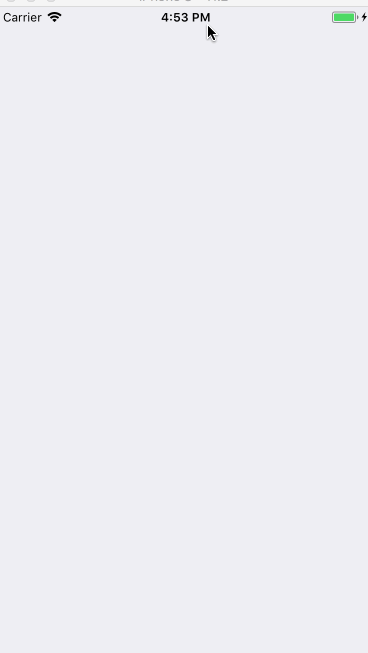空间想象能力足够的同学应该能想出来，这是绕着x轴进行的旋转，不过为啥看着反而像是沿着y轴压缩了。。。不过这里我们还是通过实验得到了一个我称为左手定则的玩意，也就是旋转方向的问题：

将左手的大拇指朝向你指定给CATransform3DMakeRotation的向量的方向，另外四指自然弯曲，弯曲的方向就是旋转的方向。

带透视效果的CATransform3D旋转

上面的效果确实是绕着x轴在旋转，之所以看起来这么奇怪，是因为我们没有添加透视效果。所谓透视，就是在平面上展现空间感的一种技术。大家在自己画一个正方体的时候都知道，如果正方体的一面不是正对着画面的话，就要画成平行四边形，这样把空间中的正方形变形成了平面上的平行四边形，给人一种立体感，这就是一种透视技术。

那么CATransform3D是如何实现透视效果的呢？CATransform3D这个矩阵是一个齐次坐标表示，我们讲齐次坐标的时候就说了，齐次坐标可以用来解决透视空间下的两条平行线相交的问题，这里就不再从计算机图形学的角度通过数学和矩阵来说明了。CATransform3D的m34（第3行第4列）的元素可以用来控制透视效果，我们只需要把它设置为−1500到−11000$-\frac{1}{500}到-\frac{1}{1000}$$-\frac{1}{500}到-\frac{1}{1000}$即可：

- (void)touchesEnded:(NSSet<UITouch *> *)touches withEvent:(UIEvent *)event
{
CALayer * layer = [CALayer layer];
layer.frame = CGRectMake(0, 0, 320, 240);
layer.position = self.view.center;
layer.contents = (__bridge id)[UIImage imageNamed:@"1.jpg"].CGImage;

CABasicAnimation * animation = [CABasicAnimation animation];
animation.keyPath = @"transform";
animation.duration = 5;
animation.removedOnCompletion = NO;
animation.fillMode = kCAFillModeForwards;

// 设置透视变换矩阵
CATransform3D perspectiveTransform = CATransform3DIdentity;
perspectiveTransform.m34 = -1.f/700;
// 将透视变换复合到旋转变换中
// 绕x轴旋转π/4
CATransform3D transform = CATransform3DRotate(perspectiveTransform, M_PI/4, 1, 0, 0);

animation.toValue = [NSValue valueWithCATransform3D:transform];
}


效果如图：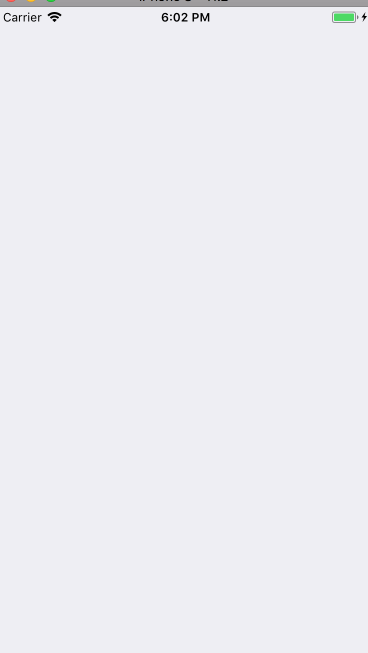这样就有明显的3D变换效果了。

基于Pan手势的3D旋转控制

旋转的问题解决了，接下来我们来看如何用pan手势来控制旋转。

总体实现思路

按照我们实践篇的思路，我们仍然先分解动画效果，分解的过程是从具体到抽象，所以我们可以这样来分解：

这是一个3D旋转效果；
这个3D旋转效果是由pan手势控制的；
旋转的方向就是手指移动的方向。

这样我们一个一个来解决。第一点，3D旋转效果我们已经知道了，用CATransform3D来实现，注意用m34来控制透视效果即可。第二点，用pan手势控制旋转效果，到这里，我们所要使用的系统API就确定了：CATransform3D和UIPanGestureRecognizer。第三点，关于旋转方向，3D旋转函数

CATransform3D CATransform3DRotate (CATransform3D t, CGFloat angle,
CGFloat x, CGFloat y, CGFloat z)

中，最后三个参数x,y,z表示一个向量v⃗=(x,y,z)$\stackrel{\to }{v}=\left(x,y,z\right)$$\vec{v} = (x,y,z)$，旋转会以该向量为轴进行旋转。所以我们在脑海里想一想，模拟一下，如果我的手指往上滑，这个视图就是“朝上旋转”的，也就是上面我们3D旋转的那个例子的示意图的效果。而此时大家想想，这个效果的“旋转轴”是什么？我们在写代码的时候传的是(1,0,0)，所以很明显这个旋转轴就是沿着x轴正方向的一个向量。也就是说我们最终要传给CATransform3D的是这个轴，而不是“旋转方向”。而旋转方向和旋转轴是有关系的：左手定则，即打开左手，大拇指指向旋转轴的方向，那么另外四指弯曲的方向就是“旋转方向”。或者我们用向量来描述的话，如果我们用D⃗$\stackrel{\to }{D}$$\vec{D}$的方向来表示旋转的方向，用C⃗$\stackrel{\to }{C}$$\vec{C}$来表示旋转轴的方向，那么C⃗$\stackrel{\to }{C}$$\vec{C}$就是D⃗$\stackrel{\to }{D}$$\vec{D}$顺时针旋转90°的结果。

旋转轴和旋转方向的问题解决了，最后一个就是手指移动的方向，这个肯定是由UIPanGestureRecognizer来提供，这样就能得到旋转方向（就是手指移动的方向），再根据旋转方向来得到旋转轴的方向，然后传给CATransform3D，就完成了一次旋转。

总结一下我们的思路：3D旋转效果用CATrasnform3D来实现；拖动手势由UIPanGestureRecognizer来实现；3D旋转方向就是手指移动的方向；手指移动的方向由UIPanGestureRecognizer提供，所以可以在回调方法里面获取到手指移动的方向；最终要传给CATransform3D的参数是旋转轴；旋转轴与手指移动方向（旋转方向）的关系是：将手指移动方向顺时针旋转90°就是旋转轴方向。

以上，我们可以通过UIPanGestureRecognizer得到手指移动方向，然后用这个方向生成一个向量，将它顺时针旋转90°得到的新向量就是我们的旋转轴向量，作为参数传给CATransform3D就行了。

通过向量计算当前手指的移动方向

接下来是最后一个难题，熟悉UIPanGestureRecognizer的同学应该知道，它的回调方法传出来的向量，即它的translationInView方法，返回的是“手指移动轨迹的起点到当前手指所在的点连成的向量”，那么我们如何来确定手指在移动的时候任意时刻手指的移动方向？我们画个图出来分析，如图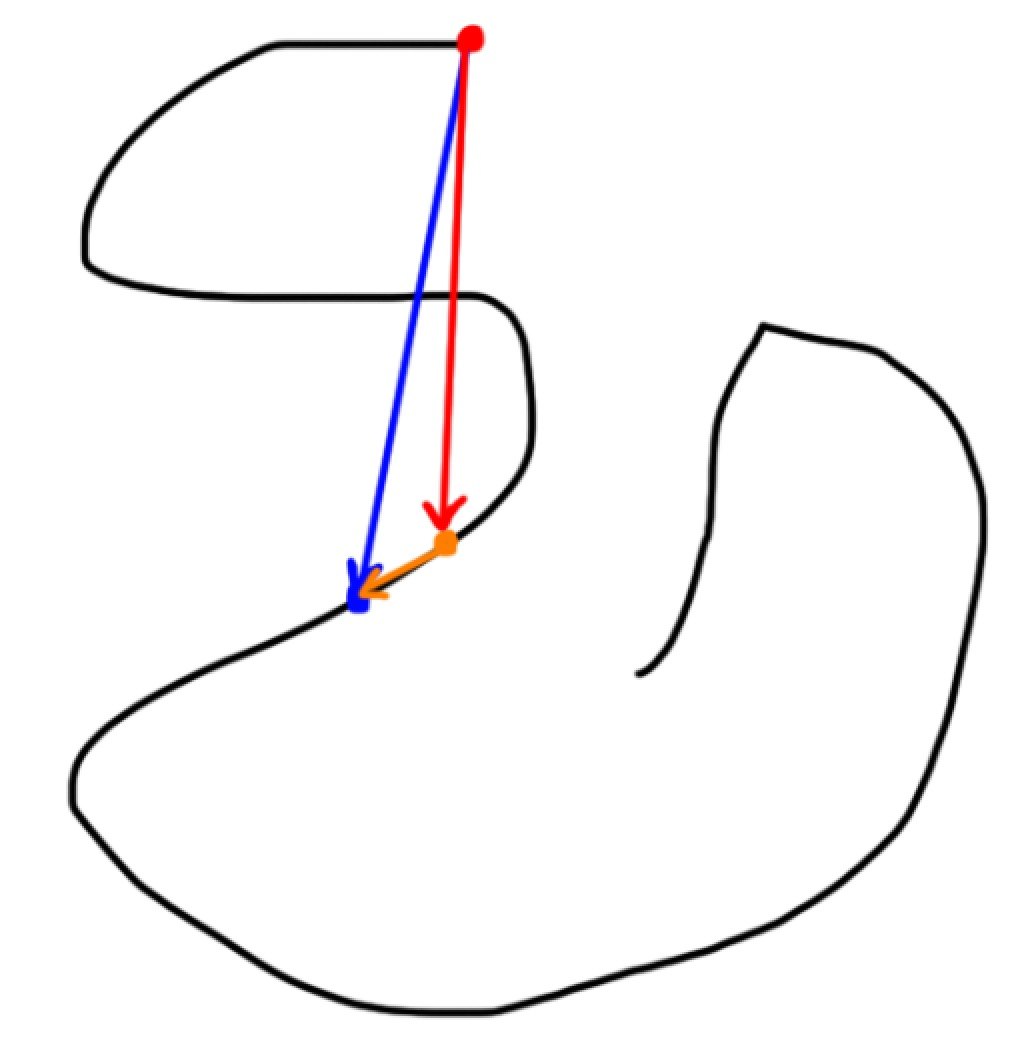假如黑色的线条就是我们手指移动的轨迹，我们在轨迹上任取一点，比如蓝色的点，当我们手指按这个轨迹移动到蓝色的点的时候，很明显，此时手指的移动方向就是蓝点的上一个点到蓝点的连线形成的方向，上一个点我用橙色来表示（为了看的更清楚橙色和蓝色我画的离得有点分开，不影响我们分析），那么橙色的箭头就是我们要找的手指的方向。然而translationInView方法返回的是当手指移动到蓝点和橙点时与起点的连线的方向，即蓝色和红色的箭头是我们已知的，我们要求出橙色箭头所代表的向量，怎么做呢？如果熟悉向量加减法的同学在这里应该能一眼就看出来了，用向量减法：

v红→+v橙→=v蓝→=>v橙→=v蓝→−v红→$\stackrel{\to }{{v}_{红}}+\stackrel{\to }{{v}_{橙}}=\stackrel{\to }{{v}_{蓝}}=>\stackrel{\to }{{v}_{橙}}=\stackrel{\to }{{v}_{蓝}}-\stackrel{\to }{{v}_{红}}$\vec{v_红} + \vec{v_橙} = \vec{v_蓝} => \vec{v_橙} = \vec{v_蓝} - \vec{v_红}

这样我们在任意时刻手指的移动方向就是：当前的translationInView减去上一次回调时传来的translationInView，我们把手势回调的代码写出来：

- (void)onPanGesture:(UIPanGestureRecognizer *)sender
{
if (sender.state == UIGestureRecognizerStateBegan) {

} else if (sender.state == UIGestureRecognizerStateChanged) {

// 获取当前手指的位移（translation）
CGPoint panTranslation = [sender translationInView:sender.view];
// 我们要的向量是手指上次所在的点到这次所在的点连成的一个向量，这是你这次手指滑动的方向，传给transform3DRotate函数的向量是垂直于这个向量的向量。而我们已知的只有这个transition，也就是手指最开始的点到手指当前点连成的一个向量（也就是手指的位移，只考虑起始点和结束点）。
// 画出图来就发现，我们要的向量就是当前向量-上一次手指的位移向量（向量减法）

// 通过这个位移生成一个向量，这就是我们当前的位移向量。
DHVector * vector = [[DHVector alloc] initWithCoordinateExpression:panTranslation];

// 用当前的位移向量-上次的位移向量得到我们手指的位移偏移量
DHVector * translateVector = [DHVector aVector:vector substractedByOtherVector:[self lastTranslation]];

// 把这个向量保存起来，下次调用这个方法的时候需要拿到这次的向量，用来做减法
// 下次再调用这个方法的时候的lastTranslation就是这次的位移向量，所以用这次的位移向量覆盖掉lastTranslation（用这次的位移向量给lastTranslation赋值）
[self setLastTranslation:vector];

// 随便计算一下单位旋转角度，也就是每次调用这个方法的时候应该旋转多少度（线性插值）

// 生成旋转向量，也就是要传给CATransform3DRotate函数的向量，它通过translateVector顺时针旋转90度（PI/2）得到
DHVector * rotateVector = [DHVector vectorWithVector:translateVector];

// 把旋转向量传给函数
self.layer.transform = CATransform3DRotate(self.layer.transform, radian, rotateVector.coordinateExpression.x,  rotateVector.coordinateExpression.y, 0);

} else if (sender.state == UIGestureRecognizerStateCancelled || sender.state == UIGestureRecognizerStateEnded) {

}
}


那么剩下的代码就比较简单了，我们把整个获取旋转轴的过程提出来写到一个方法里面去，就可以把剩下的。注意这里涉及到了手势交互，而CALayer是不支持交互的，所以这里要用UIView来做动画。

// 手指的最大位移量和当手指达到最大位移量时对应的旋转角度，用来插值计算每次手指移动应该旋转多少度
static const CGFloat maxTranslate_ = 400.f;
static const CGFloat maxRotateRadian_   =   M_PI * 2;

@interface ViewController ()

@property (nonatomic, strong) UIView * transformView;
@property (nonatomic, strong) DHVector * lastTranslation;
@property (nonatomic, assign) CGFloat transformUnit;

@end

@implementation ViewController

self.view.backgroundColor = [UIColor groupTableViewBackgroundColor];

self.transformUnit = 1.5;

}

- (void)setTransform3DWithPanTranslation:(CGPoint)translation
{
// 我们要的向量是手指上次所在的点到这次所在的点连成的一个向量，这是你这次手指滑动的方向，传给transform3DRotate函数的向量是垂直于这个向量的向量。而我们已知的只有这个transition，也就是手指最开始的点到手指当前点连成的一个向量（也就是手指的位移，只考虑起始点和结束点）。
// 画出图来就发现，我们要的向量就是当前向量-上一次手指的位移向量（向量减法）

// 通过这个位移生成一个向量。
DHVector * vector = [[DHVector alloc] initWithCoordinateExpression:translation];

// 用当前的位移向量-上次的位移向量得到我们手指的位移偏移量
DHVector * translateVector = [DHVector aVector:vector substractedByOtherVector:[self lastTranslation]];

// 把这个向量保存起来，下次调用这个方法的时候需要拿到这次的向量，用来做减法
[self setLastTranslation:vector];

// 随便计算一下单位旋转角度，也就是每次调用这个方法的时候应该旋转多少度

// 生成旋转向量，也就是要传给CATransform3DRotate函数的向量，它通过translateVector顺时针旋转90度（PI/2）得到
DHVector * rotateVector = [DHVector vectorWithVector:translateVector];

// 把旋转向量传给函数
self.transformView.layer.transform = CATransform3DRotate(self.transformView.layer.transform, radian, rotateVector.coordinateExpression.x,  rotateVector.coordinateExpression.y, 0);
}

#pragma mark - callback
- (void)onPanGesture:(UIPanGestureRecognizer *)sender
{
if (sender.state == UIGestureRecognizerStateBegan) {

} else if (sender.state == UIGestureRecognizerStateChanged) {

[self setTransform3DWithPanTranslation:[sender translationInView:sender.view]];

} else if (sender.state == UIGestureRecognizerStateCancelled || sender.state == UIGestureRecognizerStateEnded) {

}
}

#pragma mark - getter

- (UIView *)transformView
{
if (!_transformView) {
_transformView = ({

UIView * view = [[UIView alloc] initWithFrame:CGRectMake(0, 0, 320, 240)];
view.transformUnit = 1.5;
view.center = self.view.center;
view.backgroundColor = [UIColor blueColor];
view.layer.contents = (__bridge id)[UIImage imageNamed:@"1.jpg"].CGImage;
[view prepareForTransform3D];
view;

});

}
return _transformView;
}

@end


这个效果我经过简单的封装，通过category让任意视图都能通过一个方法调用来实现这一个效果，我放到了这个git仓库

总结

CoreAnimation专题的最后一篇终于结束了，整个实践篇的目的在于让大家通过我们原理篇和技巧篇的内容来解决需求中可能遇到的各种各样的动画难题，所以我在实践篇的写作中大量提及思考的过程，阅读起来可能会比较难啃，比较干涩（毕竟都是干货呢），但是我的想法是让大家读完实践篇后不仅能实现实践篇里面那么几个效果，还能拥有动画实现的基本思路（套路，即各种分解动画的思维方式），结合我们的技巧篇的各种工具，能够见招拆招，遇到什么都不怕，这样才是内力的修炼，而不是只会几个固定的招数。内力修炼的过程是比较漫长而痛苦的，我也是一步一步一个坑一个坑走过来的，希望大家都能有所收获吧！
展开全文iOS动画 计算机图形学
• 1.关于layer层的旋转方向问题  layer层遵守 左手坐标系:x向右，y向下，z朝向自己(左手大拇指顺着z轴指去，握紧拳头其余4指由x轴到y轴)  前提:当旋转角度为正数的时候逆时针旋转，当旋转角度为负数的时候顺时针...
1.关于layer层的旋转方向问题
layer层遵守 左手坐标系:x向右，y向下，z朝向自己(左手大拇指顺着z轴指去，握紧拳头其余4指由x轴到y轴)

前提:当旋转角度为正数的时候逆时针旋转，当旋转角度为负数的时候顺时针旋转
永远记着xyz的矢量方向性，如绕z轴旋转就是zxy，x-》y为正，为逆时针方向旋转，反之y-》x就是顺时针
如绕x轴旋转就是xyz，y-》z为正数，为逆时针
如绕y轴旋转就是yzx，z-》x就是正数，为逆时针
注意:(xyz无论怎么交换方向性不变永远x-》y-》z)
当绕z轴旋转，x---》y为逆时针

CATransform3D unit =CATransform3DIdentity;//单位矩阵

unit = CATransform3DRotate(unit,M_PI_4,
0,0,
1);//绕Z轴逆时针旋转

https://img-blog.csdn.net/20160818002718082?watermark/2/text/aHR0cDovL2Jsb2cuY3Nkbi5uZXQv/font/5a6L5L2T/fontsize/400/fill/I0JBQkFCMA==/dissolve/70/gravity/Center

unit = CATransform3DRotate(unit, -M_PI_4,0,0,1);//绕Z轴顺时针时针旋转】
https://img-blog.csdn.net/20160818002751160?watermark/2/text/aHR0cDovL2Jsb2cuY3Nkbi5uZXQv/font/5a6L5L2T/fontsize/400/fill/I0JBQkFCMA==/dissolve/70/gravity/Center

当绕x轴旋转，y-》z为逆时针

unit = CATransform3DRotate(unit,M_PI_4,
1,0,
0);//绕x轴逆时针

https://img-blog.csdn.net/20160818002826911?watermark/2/text/aHR0cDovL2Jsb2cuY3Nkbi5uZXQv/font/5a6L5L2T/fontsize/400/fill/I0JBQkFCMA==/dissolve/70/gravity/Center
unit = CATransform3DRotate(unit, -M_PI_4,1,0,0);//绕x轴顺时针

https://img-blog.csdn.net/20160818002856239?watermark/2/text/aHR0cDovL2Jsb2cuY3Nkbi5uZXQv/font/5a6L5L2T/fontsize/400/fill/I0JBQkFCMA==/dissolve/70/gravity/Center

当绕y轴旋转的时候，z-》x为逆时针

unit = CATransform3DRotate(unit,M_PI_4,
0,1,
0);

https://img-blog.csdn.net/20160818002938286?watermark/2/text/aHR0cDovL2Jsb2cuY3Nkbi5uZXQv/font/5a6L5L2T/fontsize/400/fill/I0JBQkFCMA==/dissolve/70/gravity/Center

unit = CATransform3DRotate(unit, -M_PI_4,0,
1,0);

https://img-blog.csdn.net/20160818002956214?watermark/2/text/aHR0cDovL2Jsb2cuY3Nkbi5uZXQv/font/5a6L5L2T/fontsize/400/fill/I0JBQkFCMA==/dissolve/70/gravity/Center


展开全文ios
•特效
• iOS开发,3D旋转效果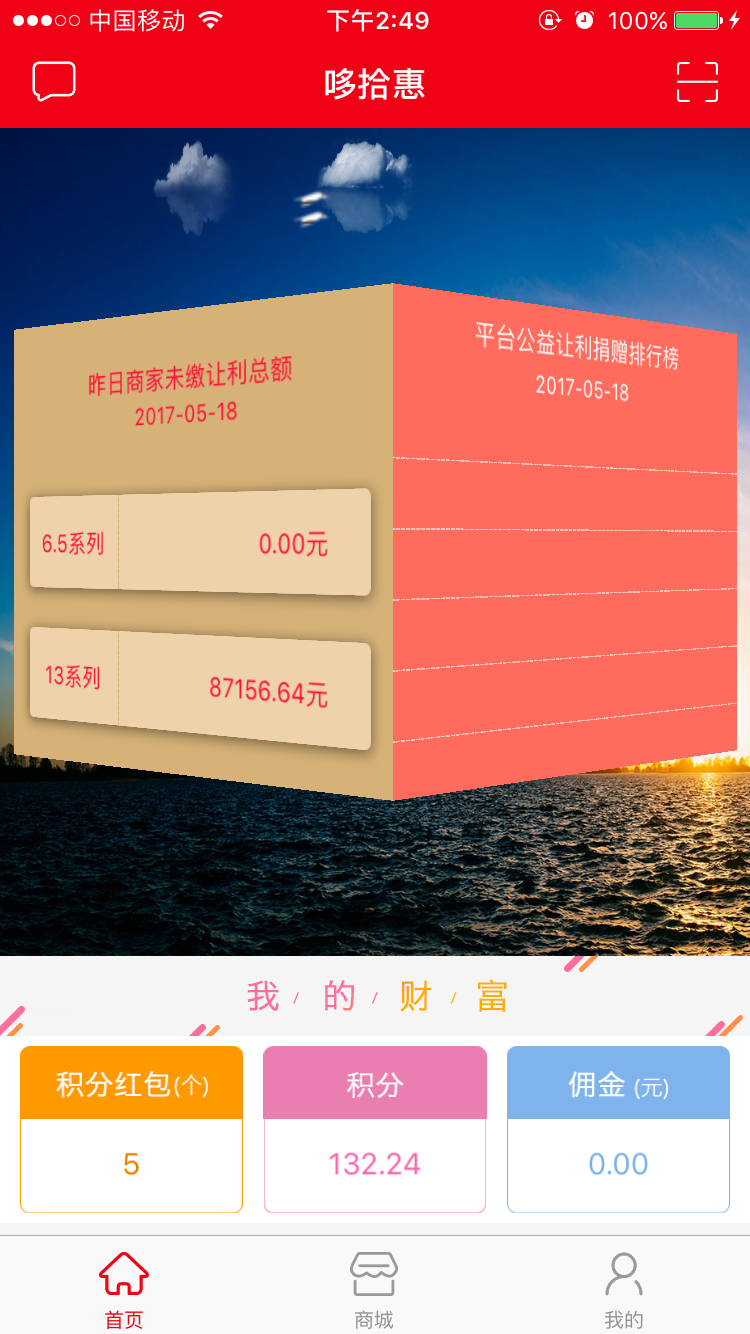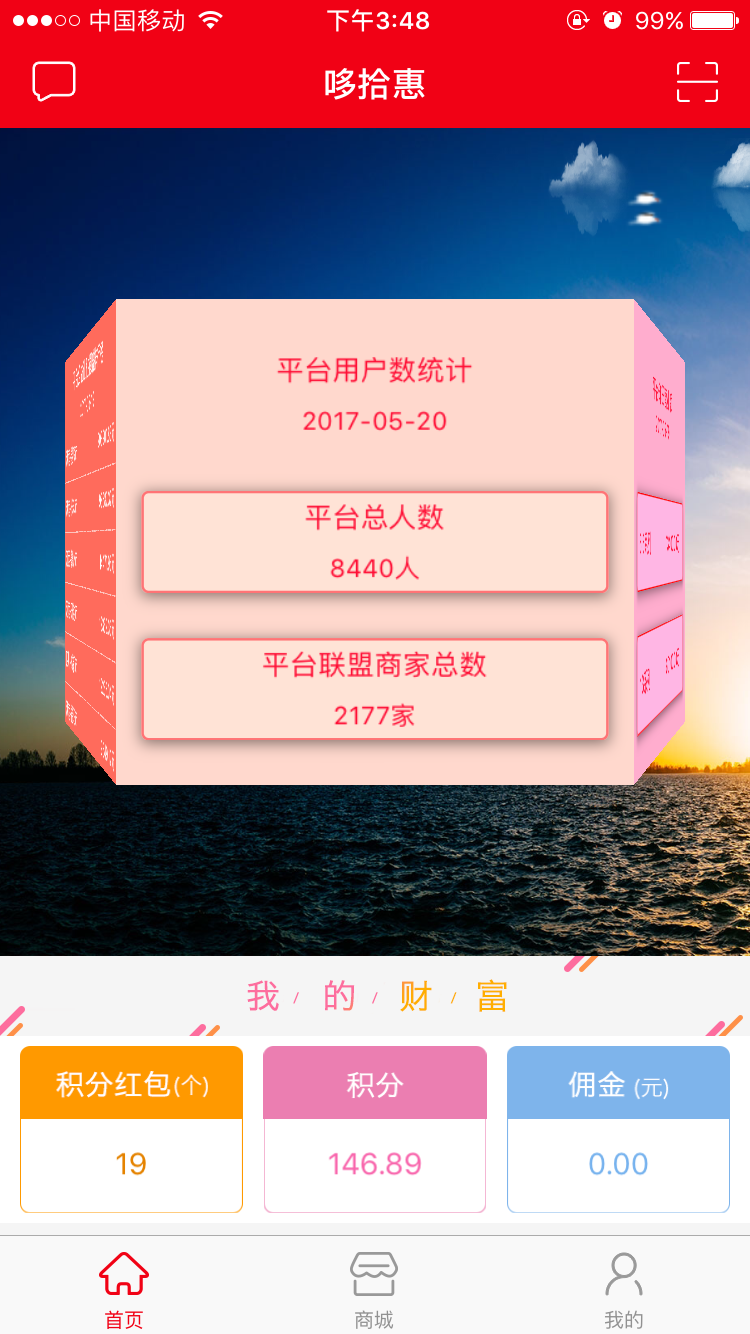立方体旋转这里用的是UICollectionView 去实现,重写UICollectionViewLayout的布局,再加入3D设置代码,当视图返回了三个以上的cell时,便可实现无缝连接的旋转立体视觉感,这里建议三个到六个之间,当返回的cell数越多,会越接近圆形!
下面贴代码:

-.第一步建立一个新的文件继承至UICollectionViewLayout 文件名为CircleLayout
.h文件为:

#import <UIKit/UIKit.h>

@interface 3DcleLayout :UICollectionViewLayout

@property(nonatomic,assign)
CGSize itemSize;//cell的尺寸

- (instancetype)initWithItemSize:(CGSize)itemSize;//重写cell尺寸方法

@end
.m文件实现

#import "3DcleLayout.h"

@implementation CircleLayout

/**

重写尺寸初始化方法

@param itemSize cell尺寸

@return返回对象

*/

- (instancetype)initWithItemSize:(CGSize)itemSize

{

if (self = [superinit])
{

_itemSize = itemSize;

}

returnself;

}

/**

返回尺寸大小

@return
返回尺寸大小

*/

-(CGSize)collectionViewContentSize

{

float width =self.collectionView.frame.size.width
*([self.collectionViewnumberOfItemsInSection:0
]+2);

float height=self.collectionView.frame.size.height;

CGSize  size =CGSizeMake(width, height);

return size;

}

- (BOOL)shouldInvalidateLayoutForBoundsChange:(CGRect)newBounds

{

returnYES;

}

//这里是关键,这设置3d效果

#pragma mark - UICollectionViewLayout

- (UICollectionViewLayoutAttributes *)layoutAttributesForItemAtIndexPath:(NSIndexPath *)indexPath

{

//3D代码

UICollectionViewLayoutAttributes* attributes = [UICollectionViewLayoutAttributeslayoutAttributesForCellWithIndexPath:indexPath];

UICollectionView *collection =self.collectionView;

float width = collection.frame.size.width;

float height = collection.frame.size.height;

float x = collection.contentOffset.x;

CGFloat arc =M_PI *
2.0f;

NSInteger numberOfVisibleItems = [self.collectionViewnumberOfItemsInSection:0];

attributes.center =CGPointMake(x+width *
0.5,height * 0.5);

//  attributes.size = CGSizeMake(width * 0.5, height - 40);

attributes.size =_itemSize;

CATransform3D transform =CATransform3DIdentity;

transform.m34 = -1.0f/700.0f;

CGFloat angle = (indexPath.row-x/width+1)/ numberOfVisibleItems * arc;

transform = CATransform3DRotate(transform, angle,0.0f,
1.0f,0.0f);

transform = CATransform3DTranslate(transform,0.f,

attributes.transform3D = transform ;

return attributes;

}

-(NSArray*)layoutAttributesForElementsInRect:(CGRect)rect

{

NSArray *arr = [superlayoutAttributesForElementsInRect:rect];

if ([arrcount] >0) {

return arr;

}

NSMutableArray* attributes = [NSMutableArrayarray];

for (NSInteger i=0 ; i < [self.collectionViewnumberOfItemsInSection:0
]; i++) {

NSIndexPath* indexPath = [NSIndexPathindexPathForItem:i
inSection:0];

}

return attributes;

}

@end

第二步:第一步已经封装好了3D效果,接下来只要去初始化UICollectionView的时候调用它即可,方法是在一个UIviewController,或者UIView上初始化一个UICollectionView,例如:

//控制上下左右边距

self.collectionView = [[UICollectionViewalloc]

initWithFrame:rect

collectionViewLayout:[[CircleLayoutalloc]

initWithItemSize:CGSizeMake(

rect.size.width *0.55,

rect.size.height -placeBank)]];

placeBank是控制3d轮播图距离上下边距的大小,rect是collectionView的frame.

到这里以后只需要在实现对应的代理方法,返回3个以上cell即可实现,cell可自行自定义,也可使用定时器做成自动旋转效果


展开全文• 今天在网到有人实现了一个旋转菜单，我用CAKeyFrameAnimation(position)和CABasicAnimation(transform.scale)也做了一个，和大家分享下， 菜单支持奇数和偶数个，点击会旋转并缩放，图如下： 实现代码：都在一...
今天在网到有人实现了一个旋转菜单，我用CAKeyFrameAnimation(position)和CABasicAnimation(transform.scale)也做了一个，和大家分享下，
菜单支持奇数和偶数个，点击会旋转并缩放，图如下：实现代码：都在一个swift即可。
1.先自定义一个UIButton类，添加两个属性
import UIKit

class CustomButton: UIButton {

var currentAngle: CGFloat = 0.0

var currentIndex: Int = 0
}

2.添加菜单类，声明几个数组分别保存按钮，按钮最开始的坐标，按钮最开始的缩放比例
class AnimationMenu: UIView {
let duration: NSTimeInterval = 0.5

var scaleArray: [CGFloat] = Array()
}3.初始化函数
    init(frame: CGRect, imageArray: [UIImage?], size: CGSize, radius: CGFloat) {
super.init(frame: frame)

}

}4.生成按钮，size已经按照他们的索引进行了相应缩放
func createMenuButtons(array: [UIImage?]) {
var pArray: [CGFloat] = Array()
let middle: CGFloat = CGFloat(array.count) / 2.0

for var i = 0; i < array.count; i++ {
let oneF = fabs(middle - CGFloat(i))

//5个menu的话里面保存的是 [2.5, 1.5, 0.5, 0.5, 1.5]
pArray.append(oneF)
}

for var i = 0; i < array.count; i++ {
let middleIndex = fabs(middle - CGFloat(i))
var scale: CGFloat = 1.0

for var j = 0; j < pArray.count; j++ {
let oneFloat = pArray[j]
if oneFloat == middleIndex {
scale = CGFloat(pow(0.7, Double(j)))
scaleArray.append(scale)
break
}
}

let button = CustomButton(frame: CGRectMake(0, 0, menuSize.width * scale, menuSize.height * scale))
button.setBackgroundImage(array[i], forState: UIControlState.Normal)
button.currentIndex = i
button.tag = 101 + i

}
}
5.把按钮按照圆形路径排列
func addMenuViews() {
let circle = CAShapeLayer()
let circleCenter = CGPointMake(CGRectGetMidX(bounds), CGRectGetMidY(bounds))

circle.fillColor = UIColor.clearColor().CGColor
circle.strokeColor = UIColor.grayColor().CGColor
circle.lineWidth = 2.0

circle.path = UIBezierPath(arcCenter: circleCenter, radius: radius, startAngle: 0.0, endAngle: CGFloat(M_PI * 2), clockwise: true).CGPath
//注掉这行可以去除那个圆

//开始角度为最下面，也就是90度
let originStartAngle = CGFloat(M_PI * 0.5)

//顺时针从圆的最下面一个点开始平均排列
for var i = 0; i < menuArray.count; i++ {
let averageAngle = M_PI * 2 / Double(menuArray.count)

//正余弦求button中心点到圆中心点距离
let dX = CGFloat(sin(averageAngle * Double(i))) * radius
let dY = CGFloat(cos(averageAngle * Double(i))) * radius

let point = CGPointMake(circleCenter.x - dX, circleCenter.y + dY)
button.center = point

let endAngle = originStartAngle + CGFloat(Double(i) / Double(menuArray.count) * M_PI * 2.0)
button.currentAngle = endAngle
button.currentIndex = i
}

}
6.点击最大的按钮时触发事件，否则转动这个按钮到最大
    func buttonClicked(button: CustomButton) {
//currentIndex为0的是最大的那个
if button.currentIndex == 0 {
let reallyIndex = button.tag - 100
} else {
showMoveAndScaleAnimation(button)
}
}7.旋转+缩放动画实现
    func showMoveAndScaleAnimation(button: CustomButton) {
//圆中心点
let circleCenter = CGPointMake(CGRectGetMidX(bounds), CGRectGetMidY(bounds))

let moveCount = menuArray.count - button.currentIndex

for var i = 0; i < menuArray.count; i++ {

let arcPath = CGPathCreateMutable()

//结束角度
let endAngle = button.currentAngle + CGFloat(M_PI * 2.0 / Double(menuArray.count) * Double(moveCount))

let nextIndex = (button.currentIndex + moveCount) % menuArray.count

let positionAnimation = CAKeyframeAnimation(keyPath: "position")
positionAnimation.path = arcPath
positionAnimation.calculationMode = "paced"

let scaleAnimation = CABasicAnimation(keyPath: "transform.scale")
scaleAnimation.fromValue = scaleArray[button.currentIndex]
scaleAnimation.toValue = scaleArray[nextIndex]

//组合两个动画
let animationGroup = CAAnimationGroup()
animationGroup.animations = [positionAnimation, scaleAnimation]
animationGroup.fillMode = kCAFillModeForwards
animationGroup.removedOnCompletion = false
animationGroup.duration = NSTimeInterval(CGFloat(moveCount) / 4.0) + duration
animationGroup.autoreverses = false

button.currentIndex = nextIndex
button.currentAngle = endAngle

}
}测试代码：
        let array = [UIImage(named: "image1"), UIImage(named: "image2"), UIImage(named: "image3"), UIImage(named: "image4"), UIImage(named: "image5")]
let progessView = AnimationMenu(frame: CGRectMake(50, 100, 300, 300), imageArray: array, size: CGSizeMake(100, 100), radius:90)
view.addSubview(progessView)

over,具体的实现并不难，我写的比较啰嗦，请大家见谅。下一篇准备用swift写一个3D的立方体。


展开全文swift
• 在测试Unity3D ZXing代码时，调用iOS摄像头的效果总不令我满意，你会发现用WebCamTexture调用的摄像头画面跟iOS自带相册调用的效果不一样，焦距有点问题。虽然这不影响ZXing的调用，但我还是搜了下，解决了这个问题...unity3d ios 全屏 zxing
• CGPathRef aPath; aPath = CGPathCreateWithEllipseInRect(CGRectMake(0, 0, 200, 200), NULL); [CATransaction begin]; CAKeyframeAnimation * arcAnimation = [CAKeyframeAnimation animatioiOS
• 3D旋转 仿抖音小视频点赞动画 高德地图SDK二次封装，完美适配iOS11. iOS图表二次定制 - 波浪折线图 iOS图表二次定制 - 柱状图 CollectionView实现瀑布流 基于AVPlayer的自定义视频...ios源码
• 如果还没看前一篇，可以移驾看看：ios 3D引擎 SceneKit 开发(4) –关于旋转的几点问题(1)上一篇我们用CABasicAnimation 来模拟了太阳-地球-月球的天体运动。其中月球绕太阳运动和月球绕地球运动都可以看做一个点绕另...ios 3d引擎
• iOS开发14-iOS CATransform3DRotate旋转动画    有问题请联系博主，邮箱：nathanlee1987@aliyun.com 1、围绕X/Y/Z轴旋转 - (void)viewDidLoad { [super viewDidLoad]; self.myIMV=[[UIImageView ...ios开发
• Unity3diOS的交互（1） 今天我们介绍Unity3diOS交互第一部分：iOS传消息到Unity3d中。下面我们开始吧： 1. 首先用Unity3d创建一个Plain，并调整好摄像机的角度以及光源的位置，如下所示： ...ios action vector xcode interface
• 通过使用Category给UIView添加了能够从四边从内向外3D旋转，其实如果有多种的话欢迎到我的github项目（https://github.com/beautylim/Objective-C-mainViewRotate）里folk， 使用的方法是： topView.topRotate()....
• 在 iPhone 应用里，有时我们想强行把显示模式... 目前横竖屏旋转方式分为手动和自动 手动旋转 手动旋转也有2种方式，一种是直接设置 UIDevice 的 orientation，但是这种方式不推荐，上传appStore有被拒的风险：iOS横屏xcode